# Concise Selina Solutions for Class 9 Maths Chapter 15 Construction of Polygons

Selina solutions for Class 9 Maths Chapter 15 Construction of Polygons are provided here. Class 9 is one of the most important phase in a student’s life as the concepts which are taught in Class 9 are to be continued in Class 10. To score good marks in Class 9 Mathematics examination, it is advised to solve questions provided in each exercise of all the chapters in the Selina book. These Selina solutions for Class 9 Maths help the students in understanding the concepts given in a better way. Download PDF of Class 9 Maths Chapter 15 Selina Solutions from the given links.

### Download PDF of Selina Solutions for Class 9 Maths Chapter 15:-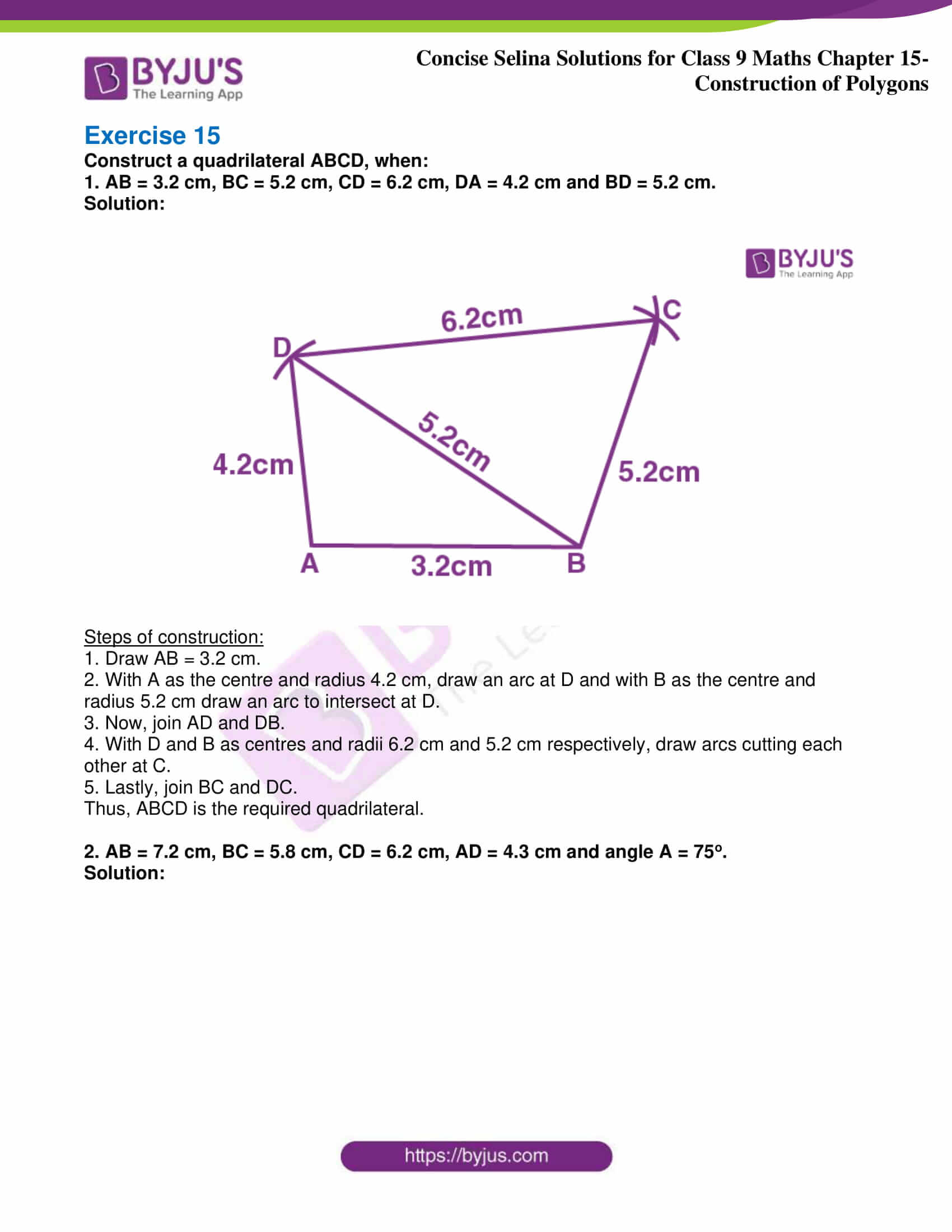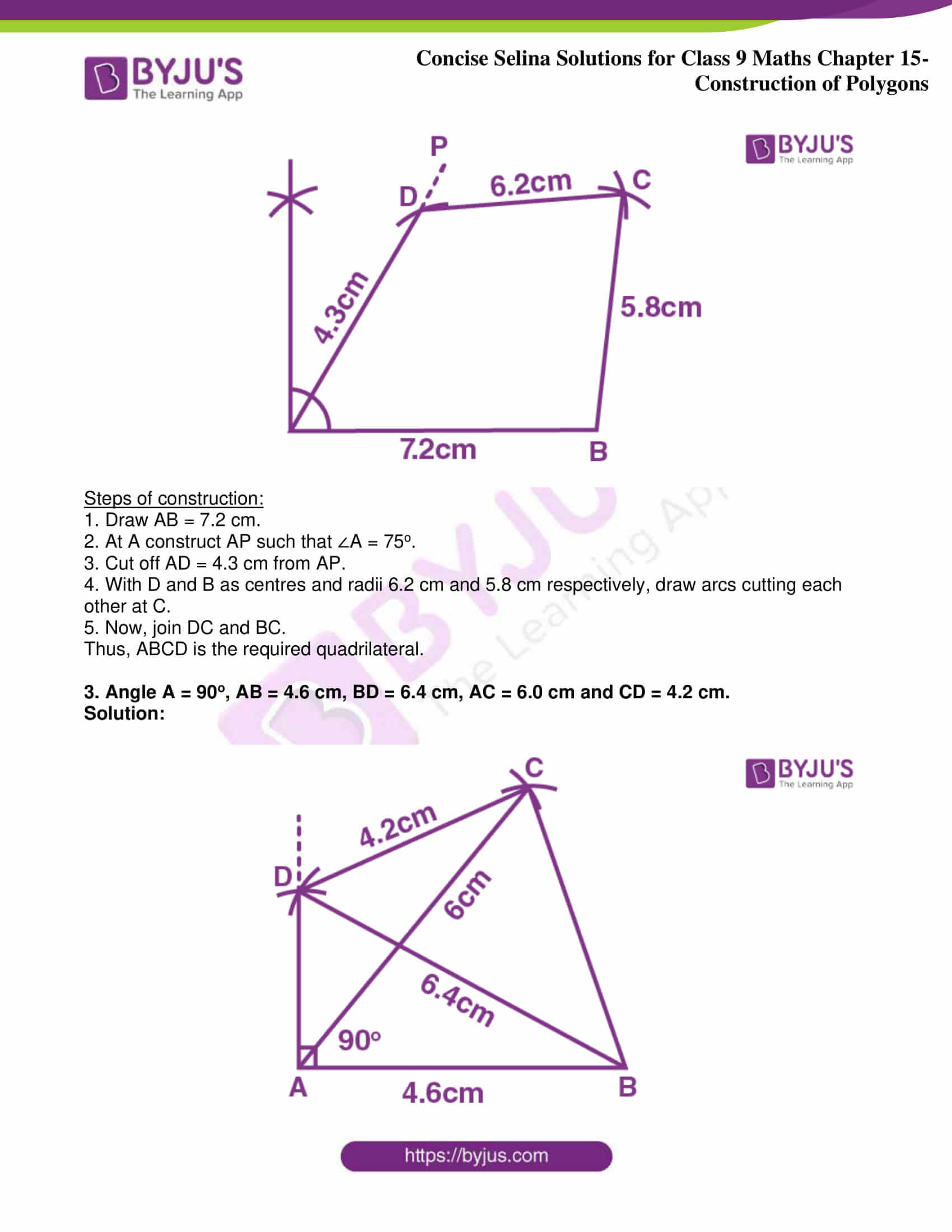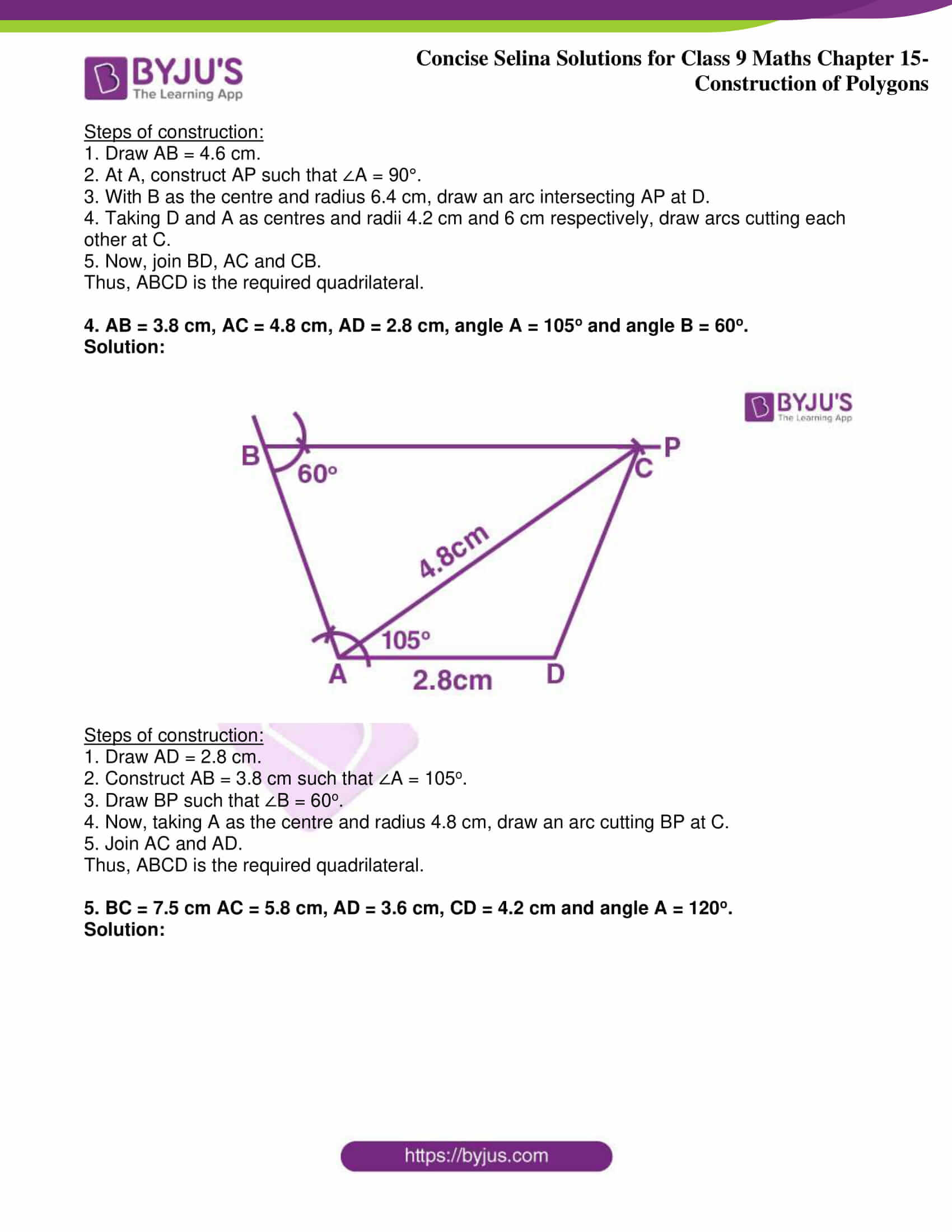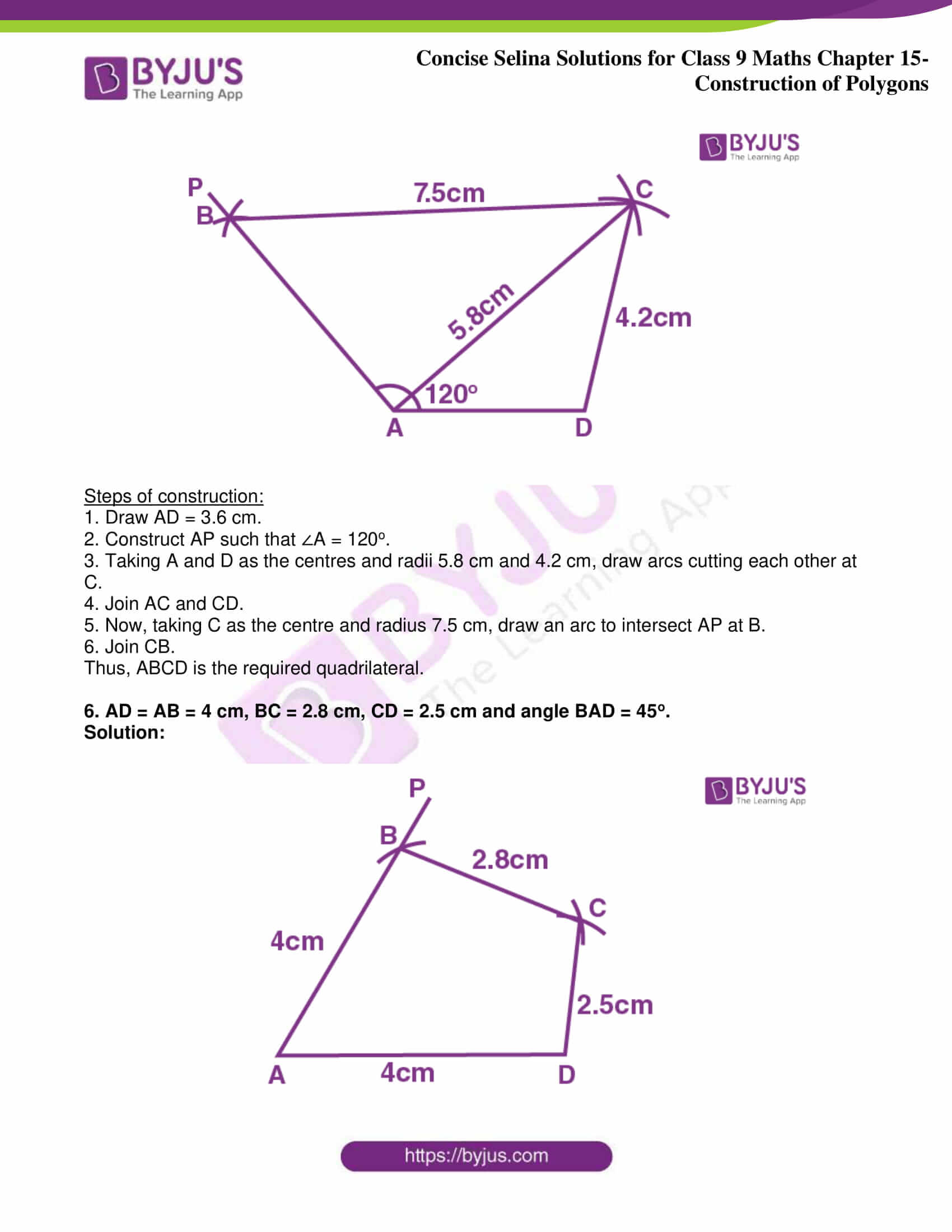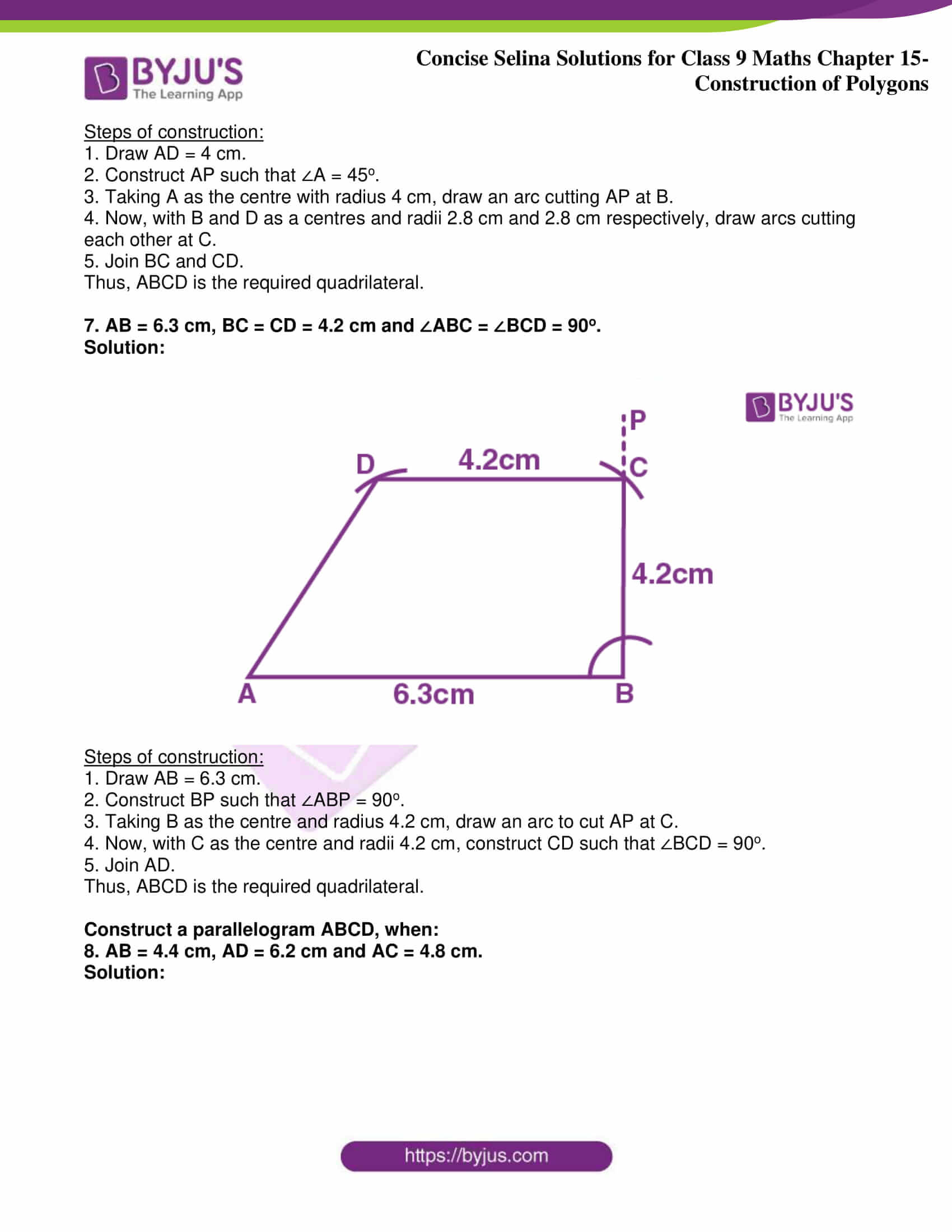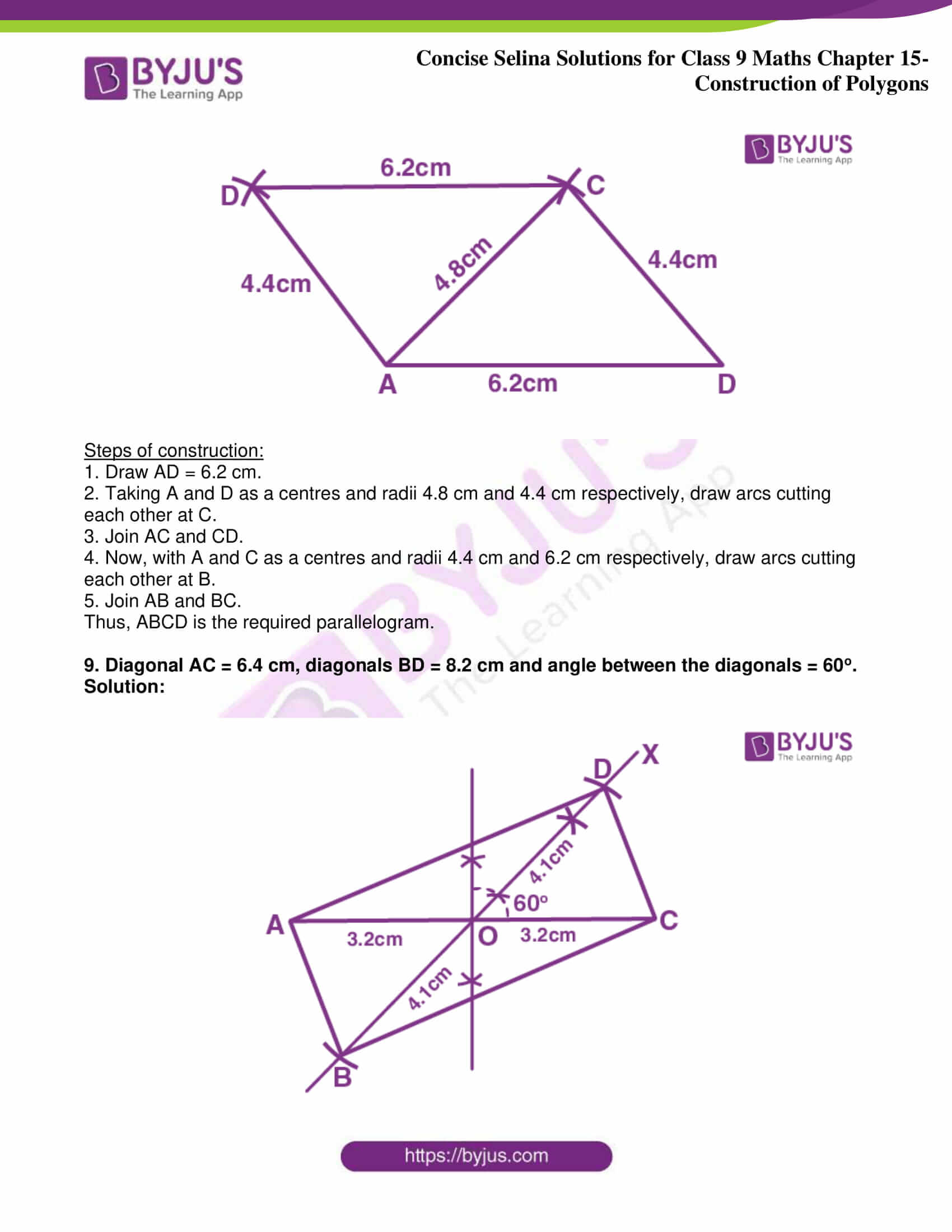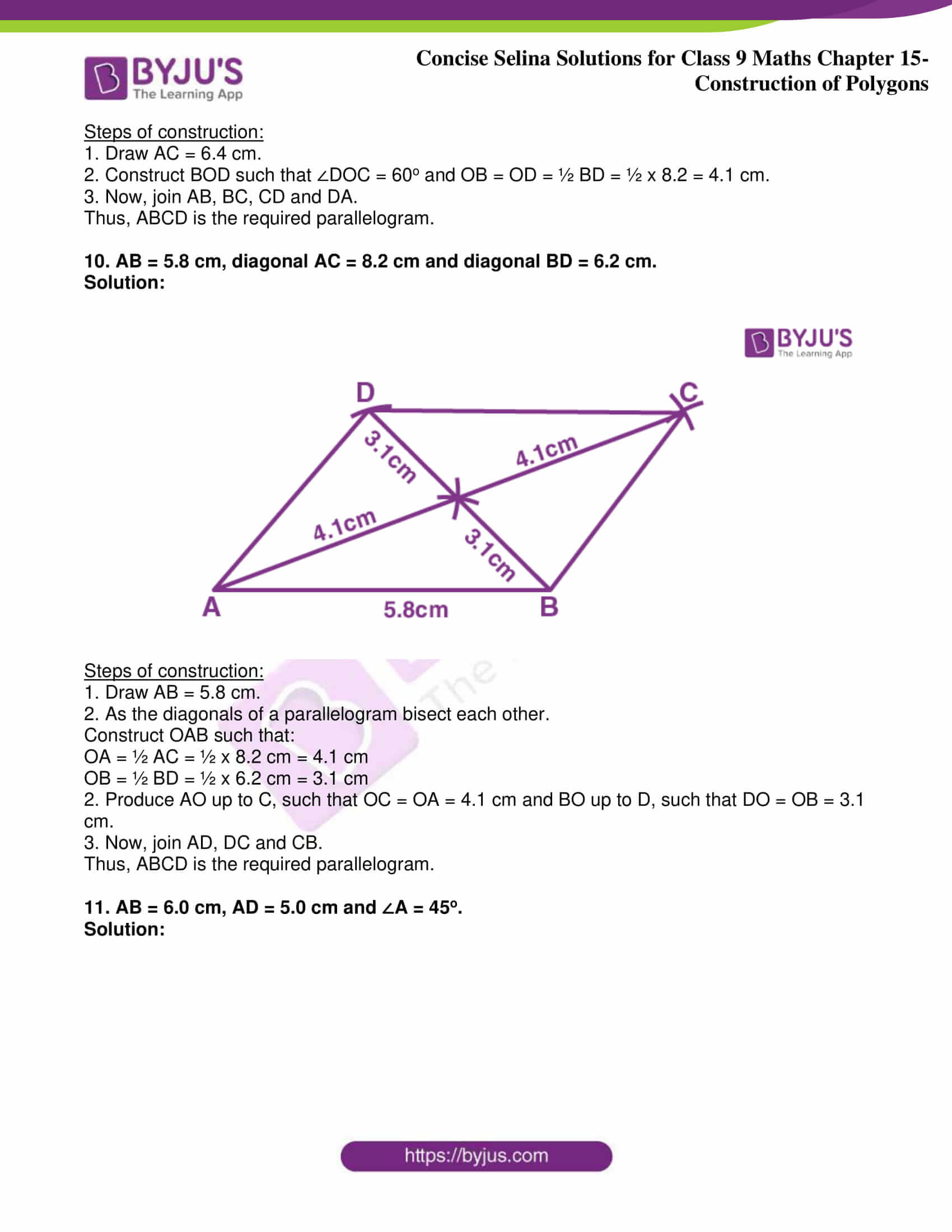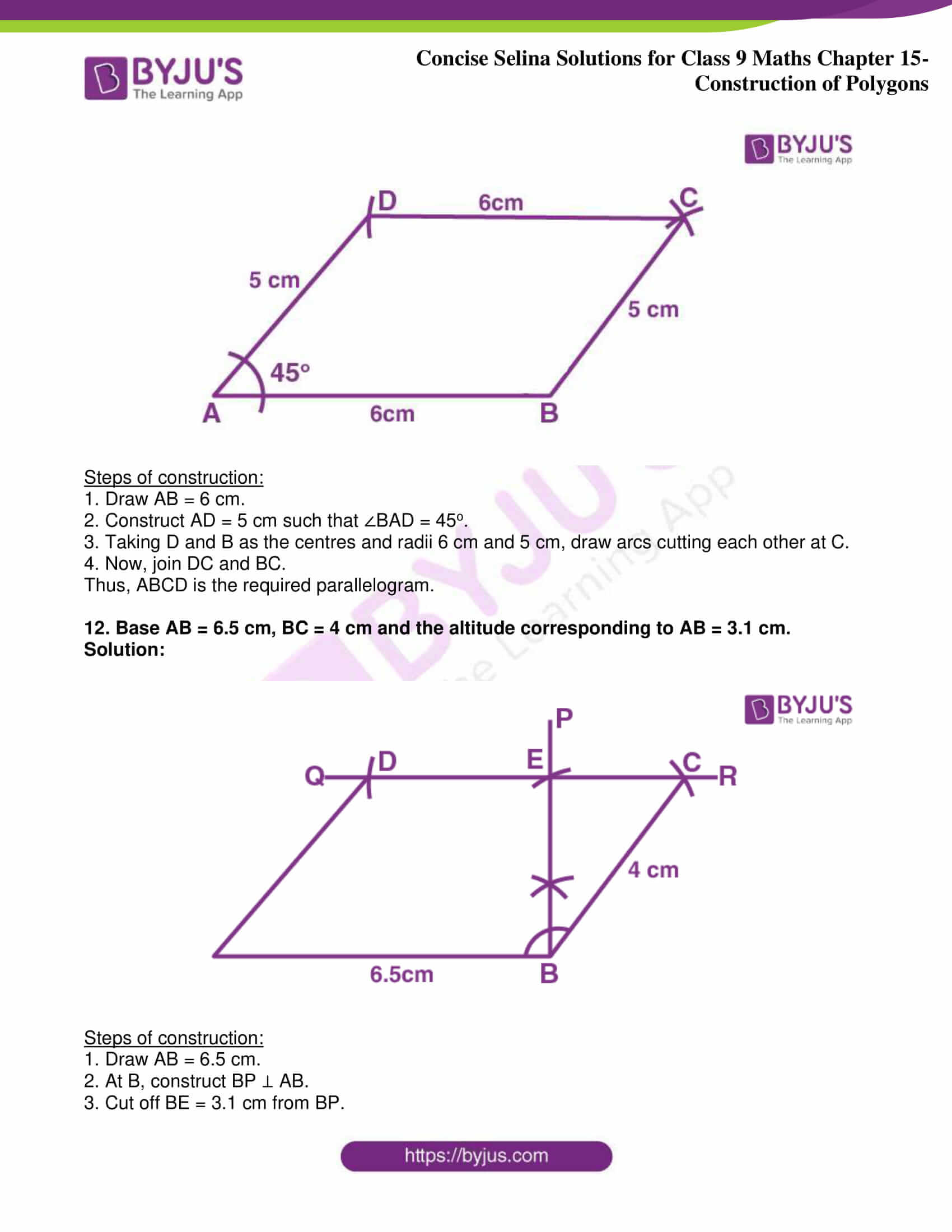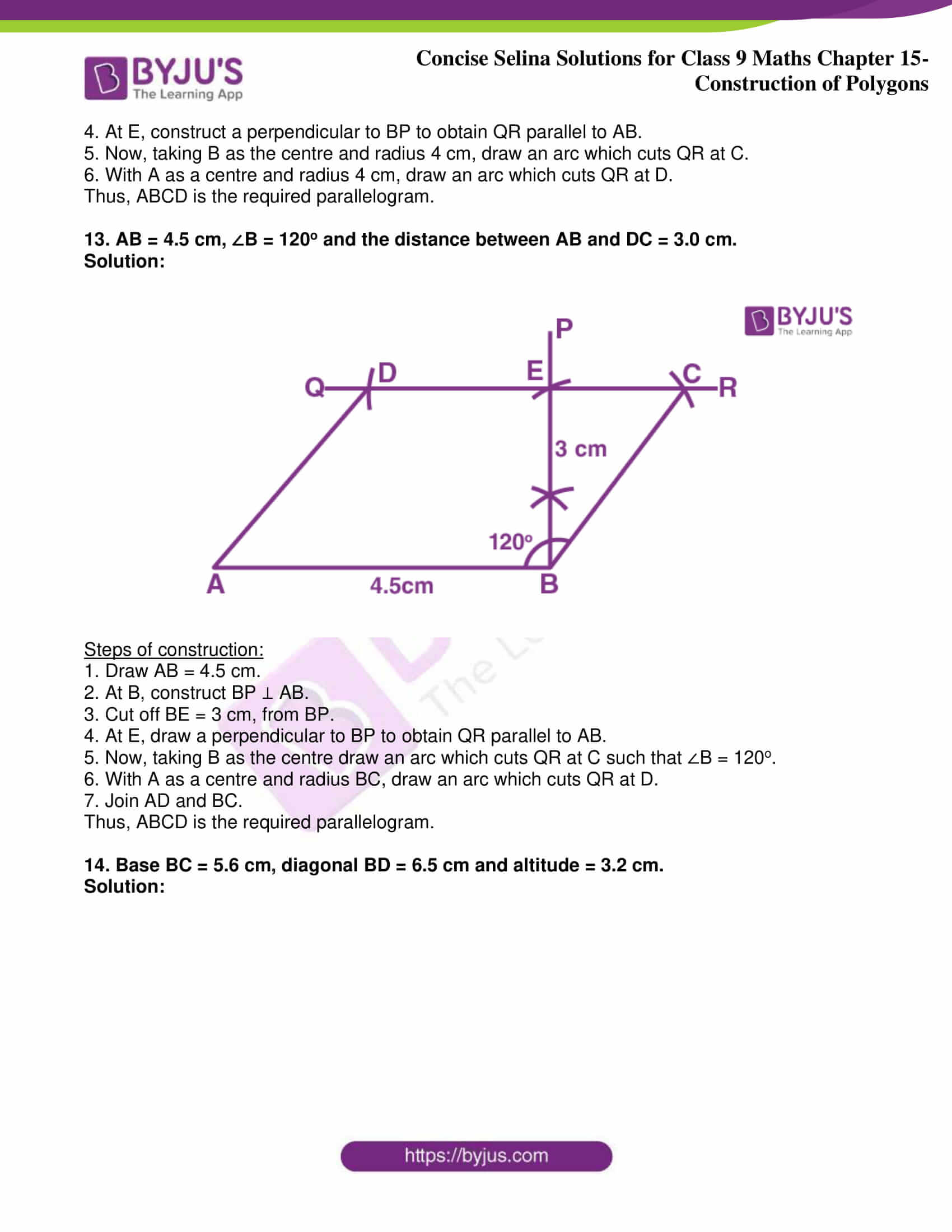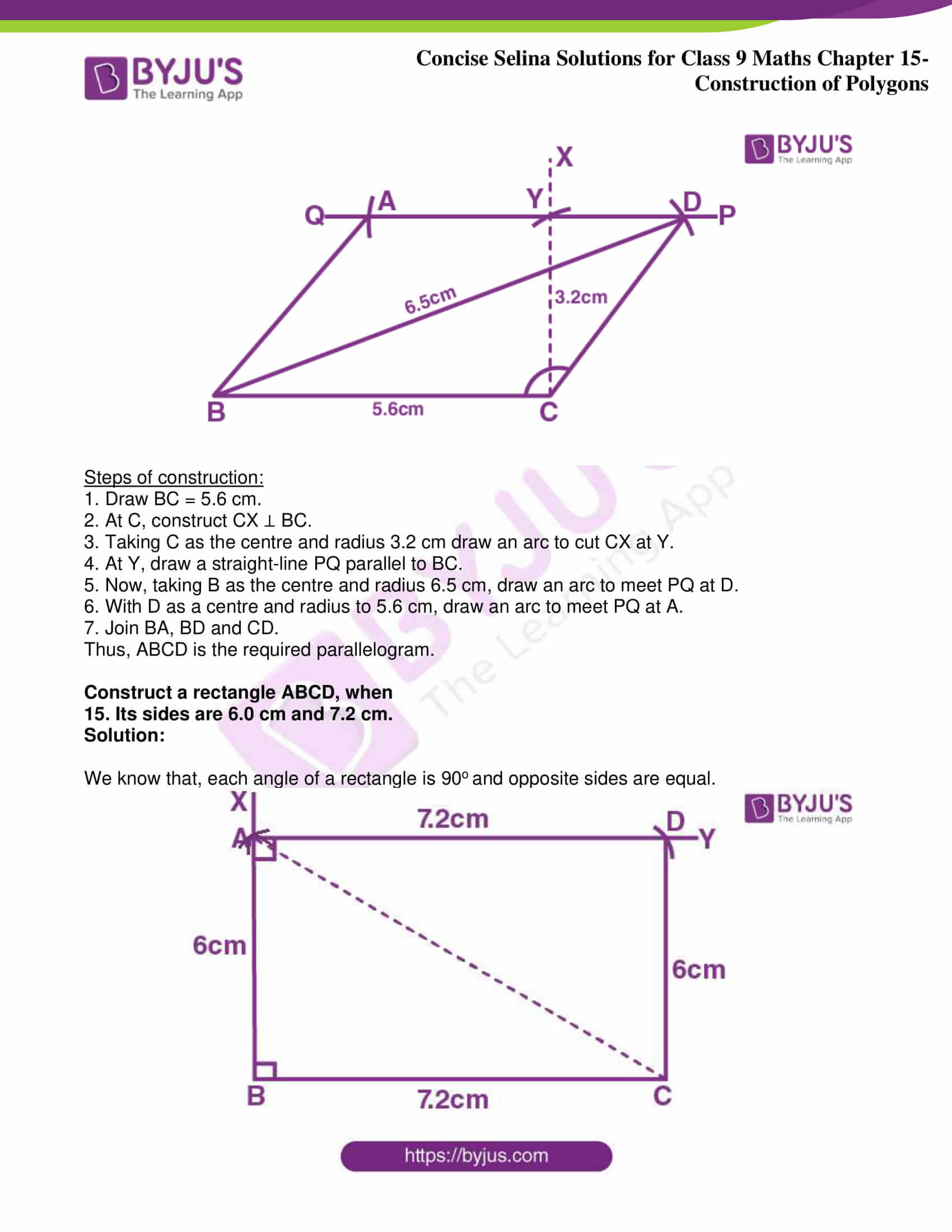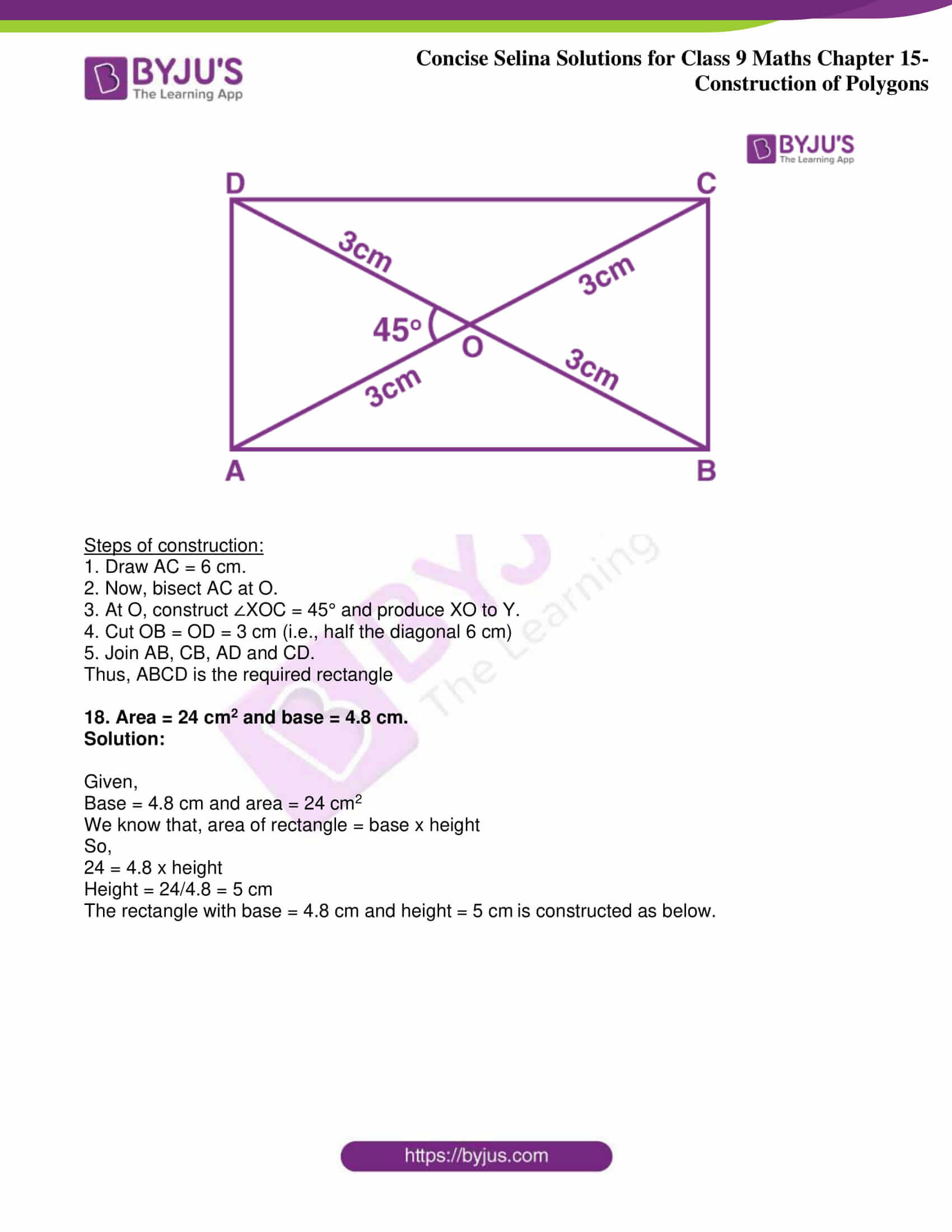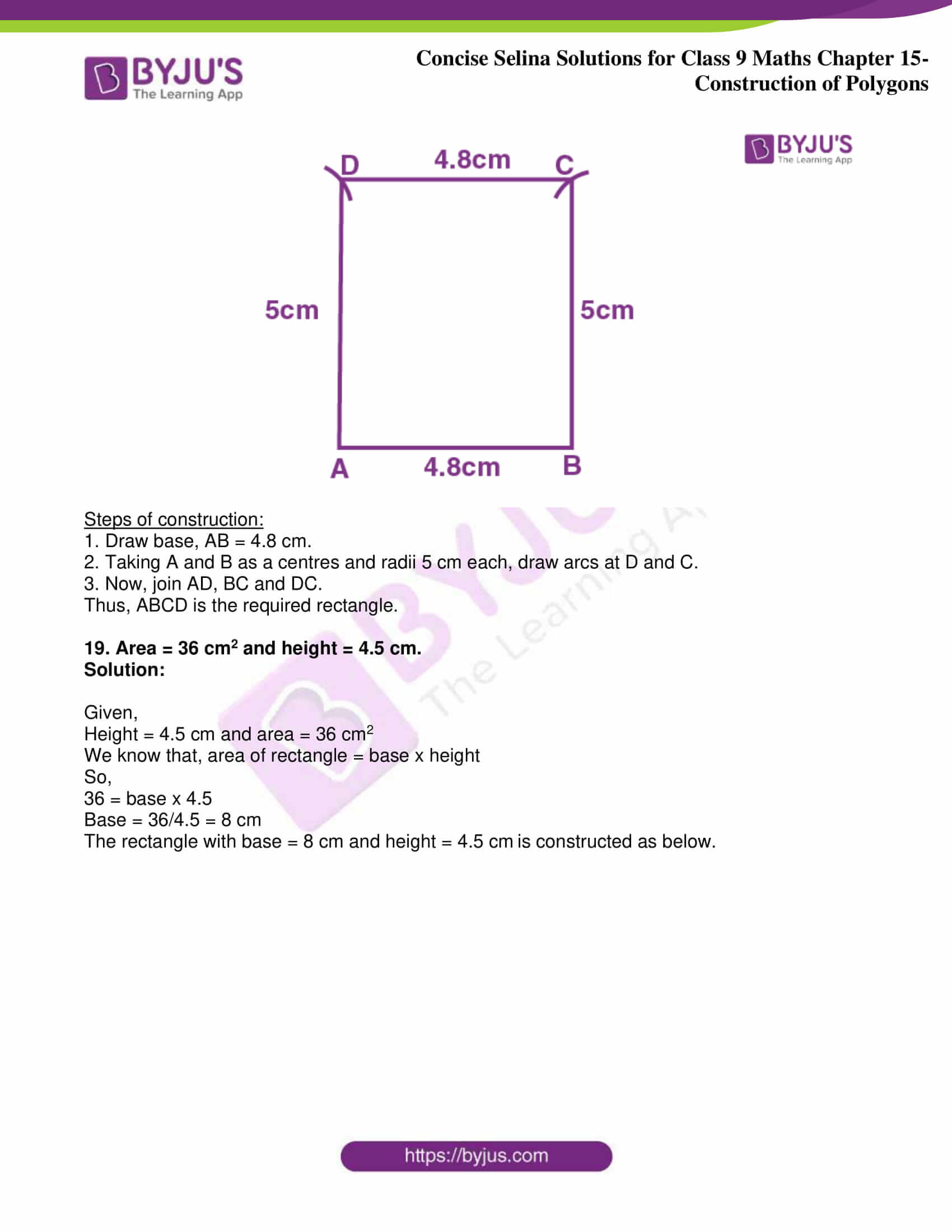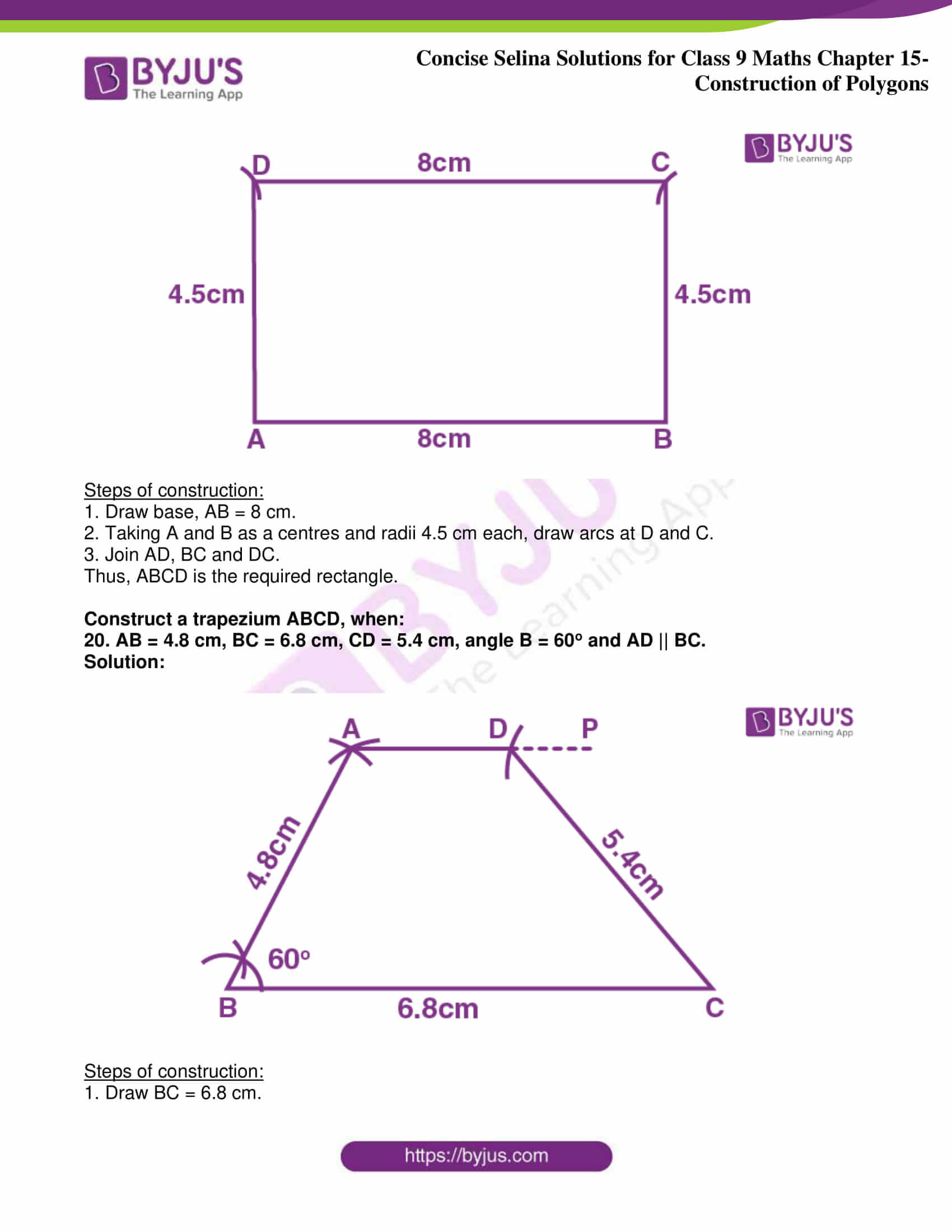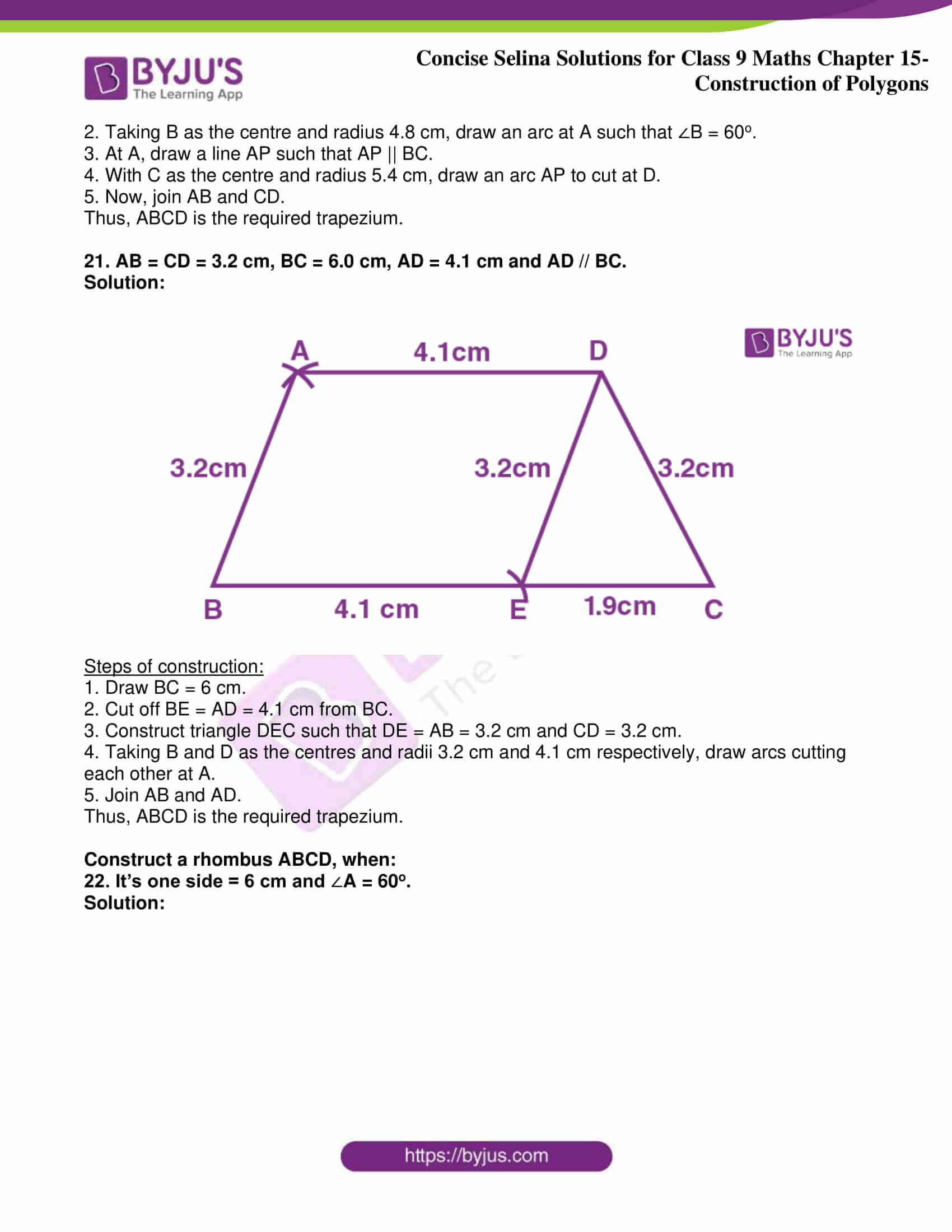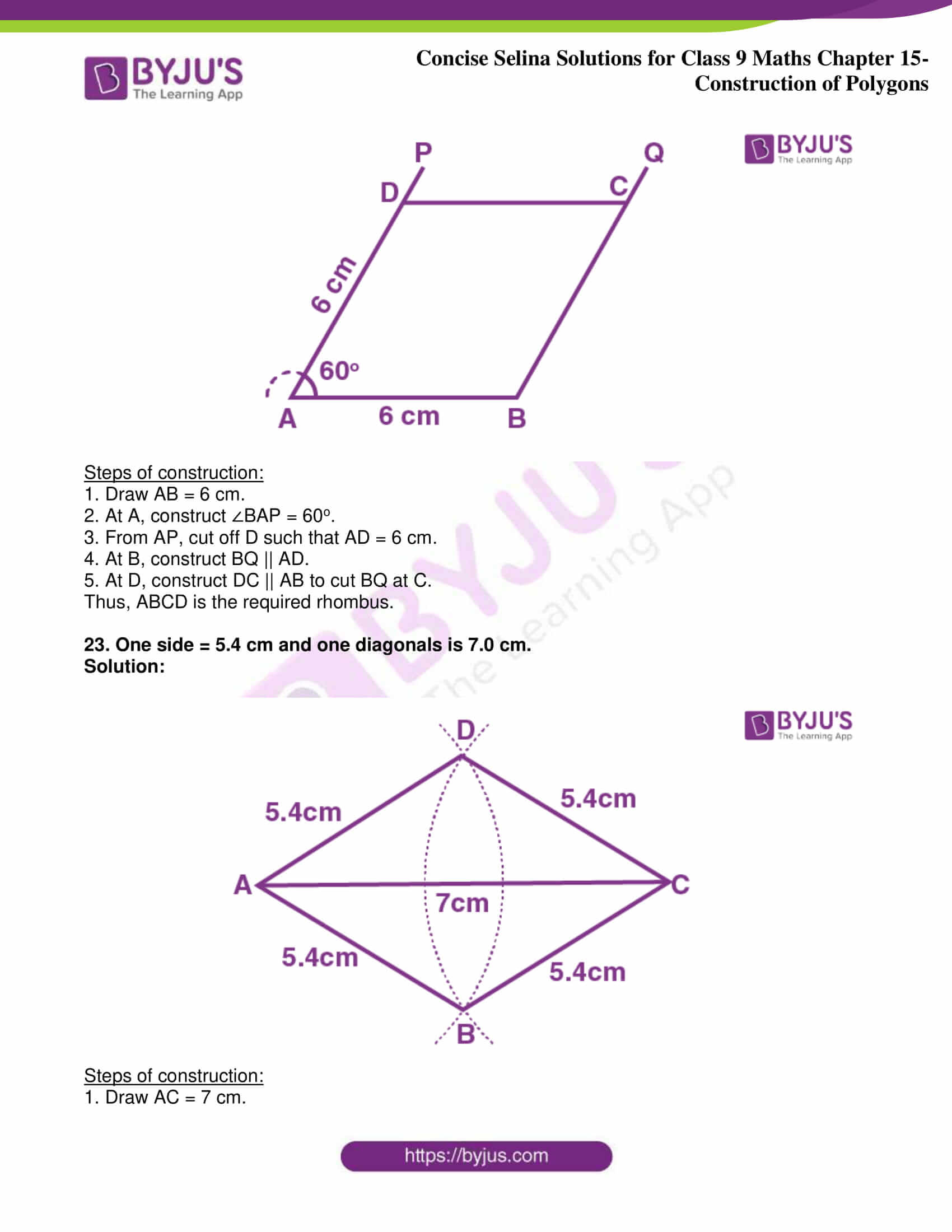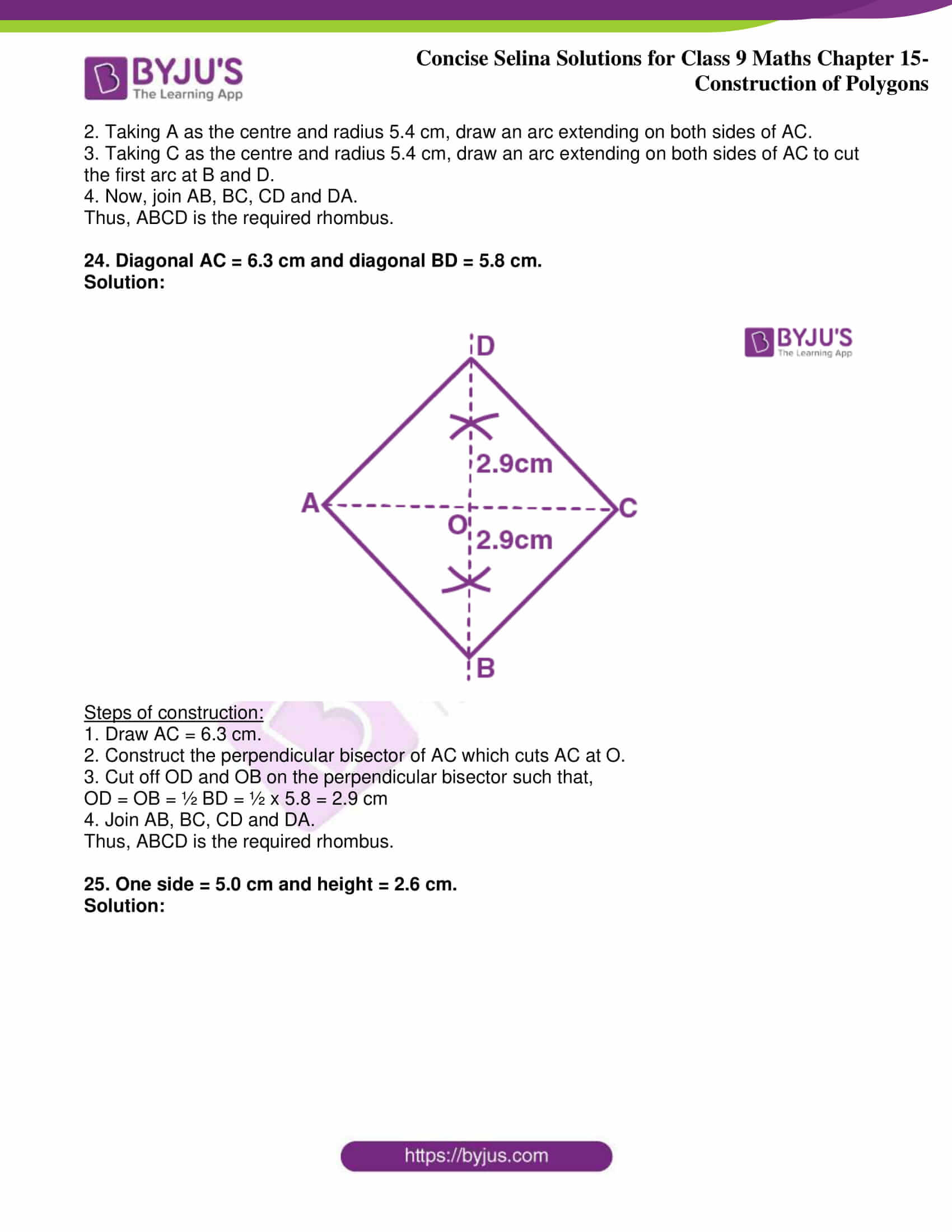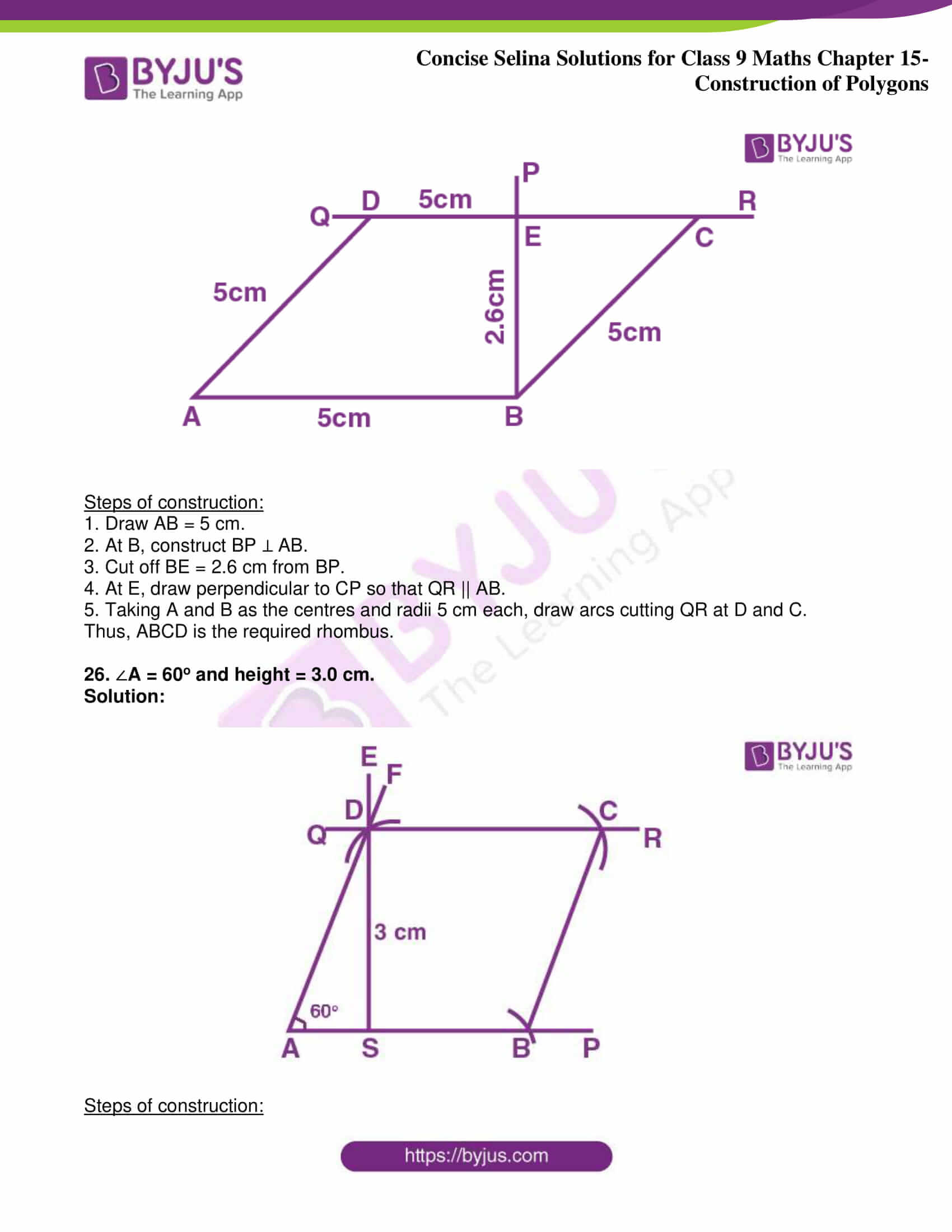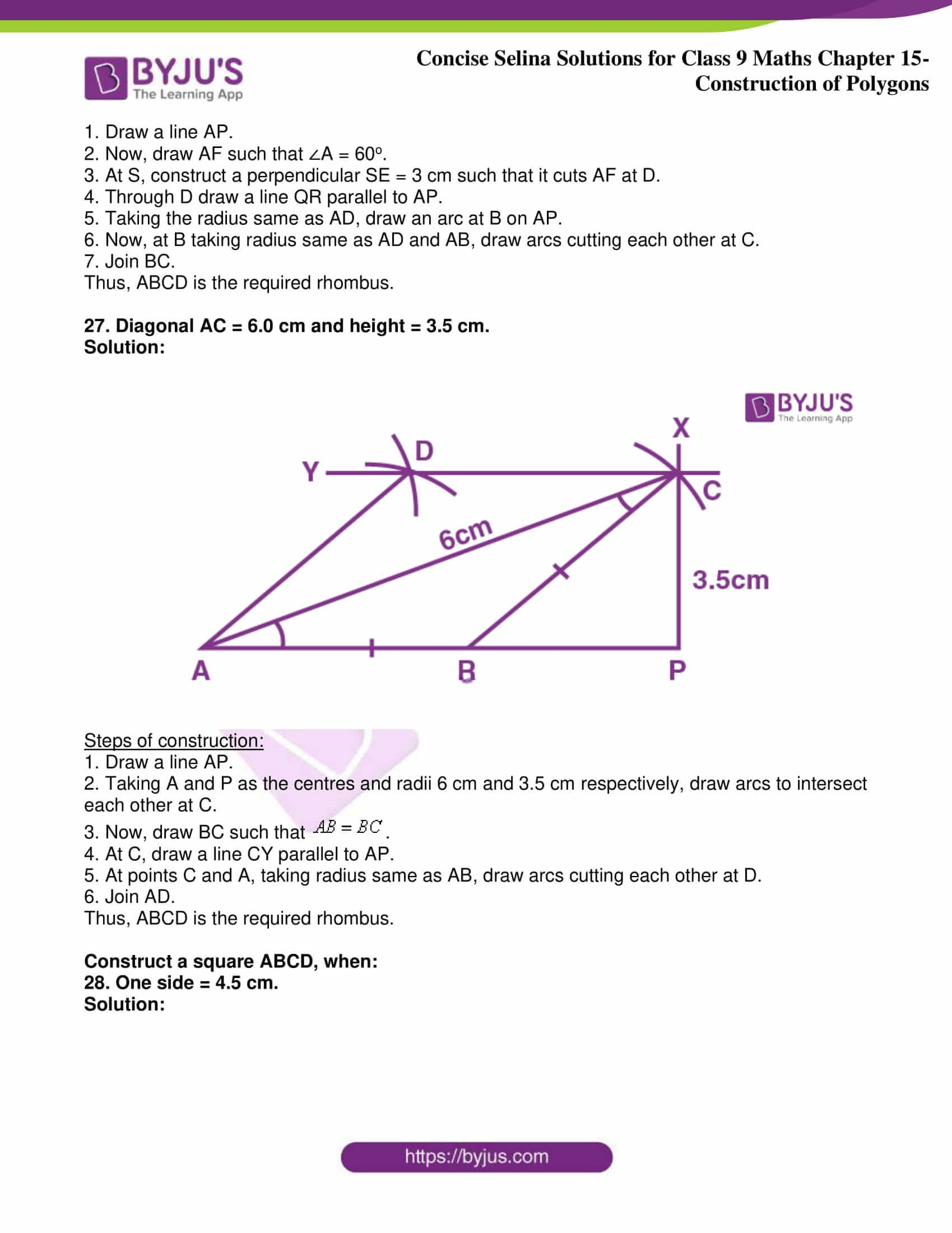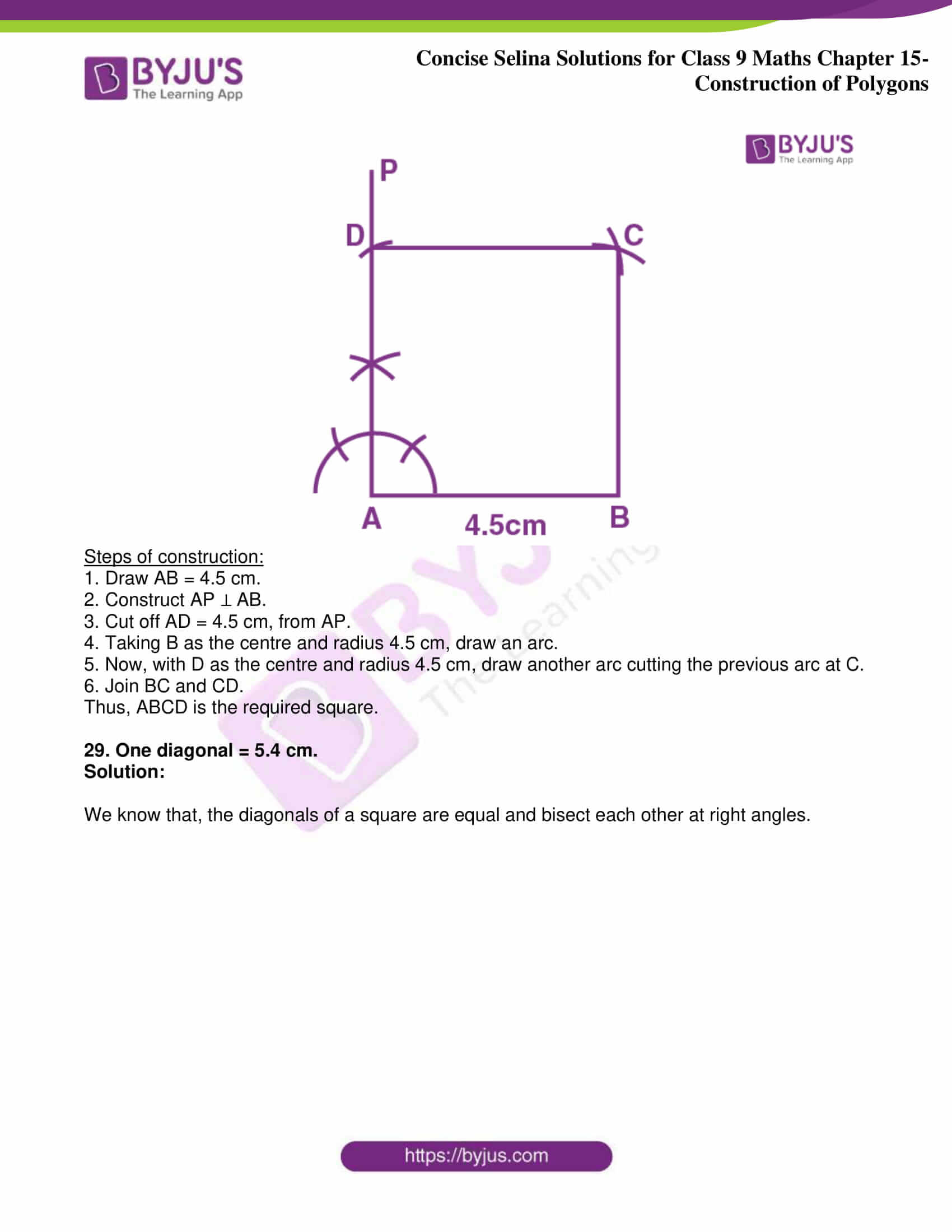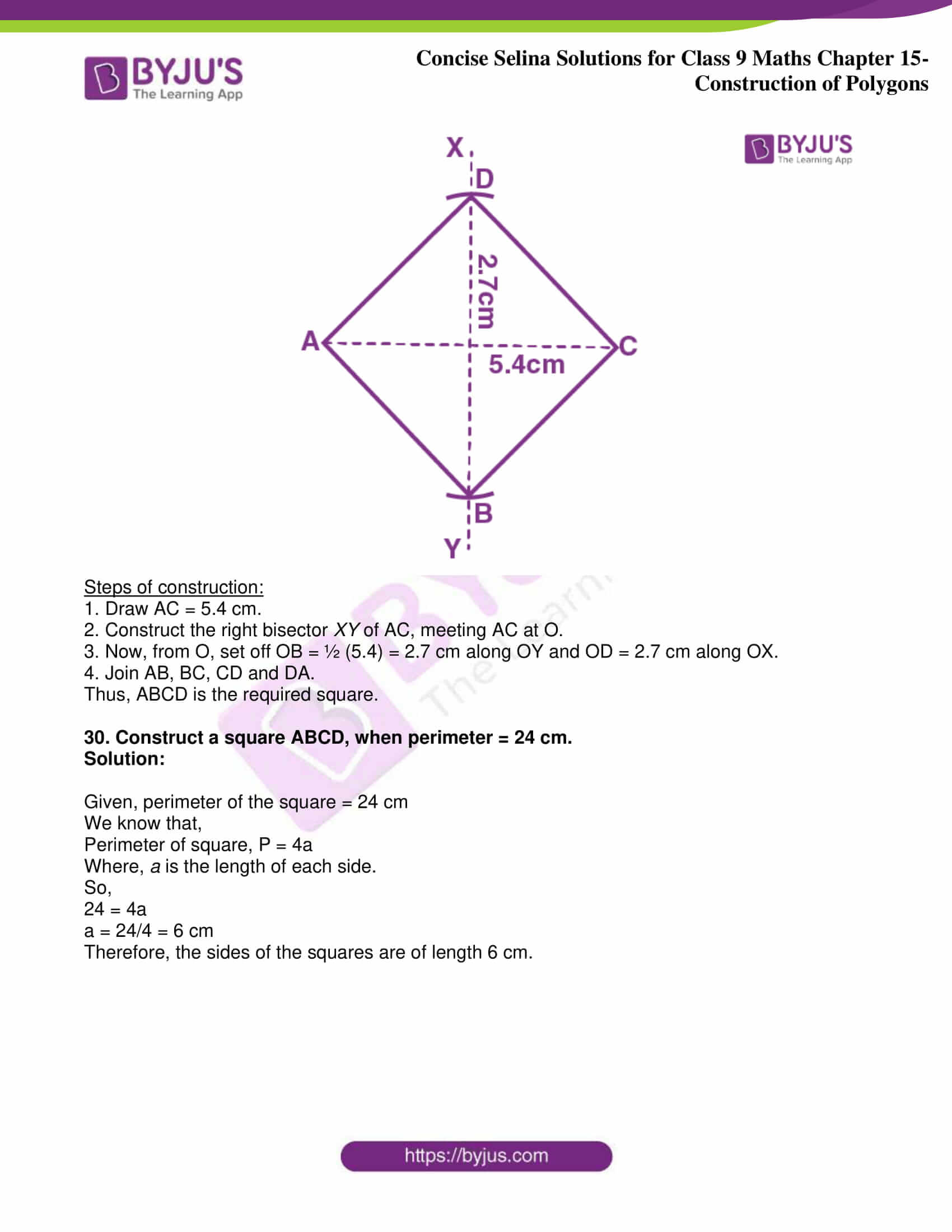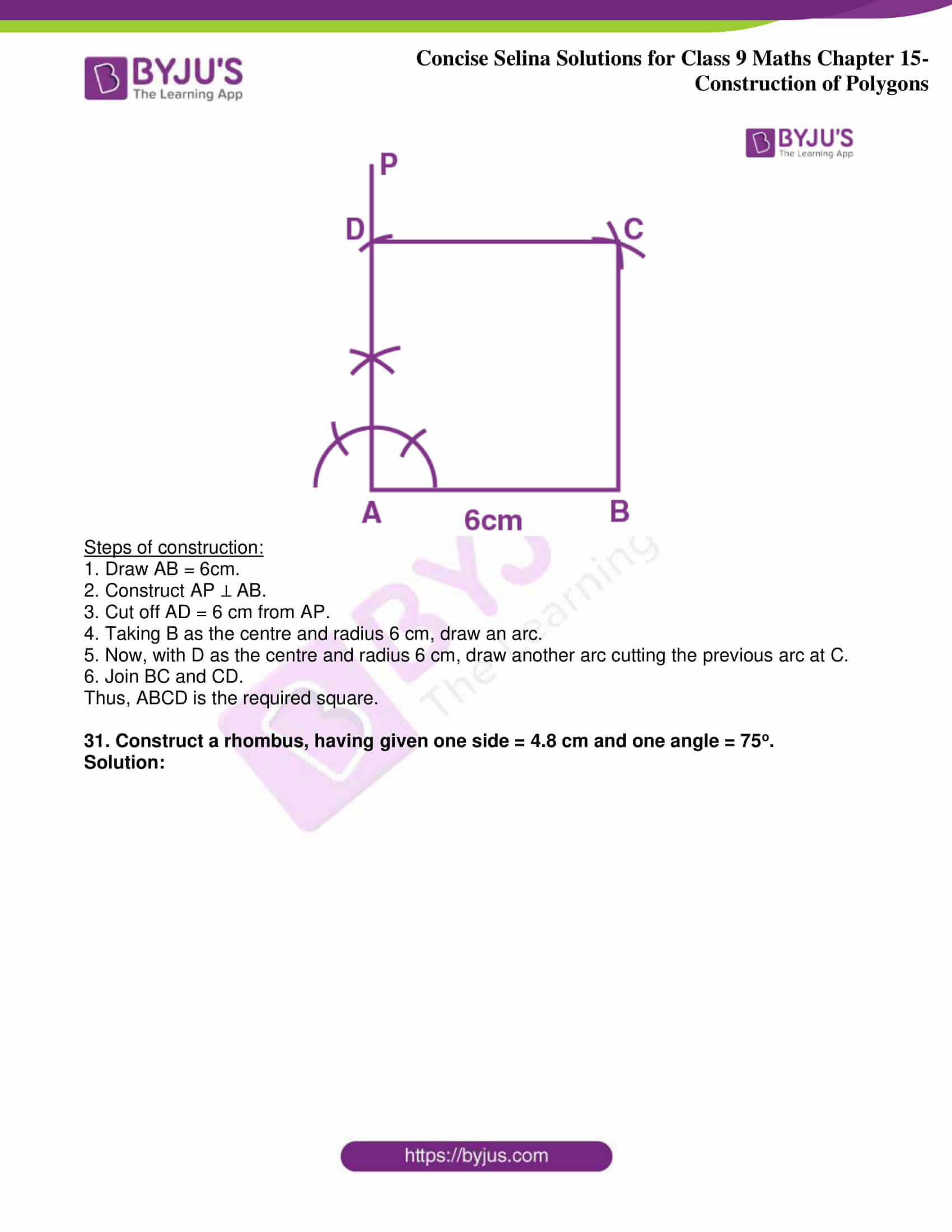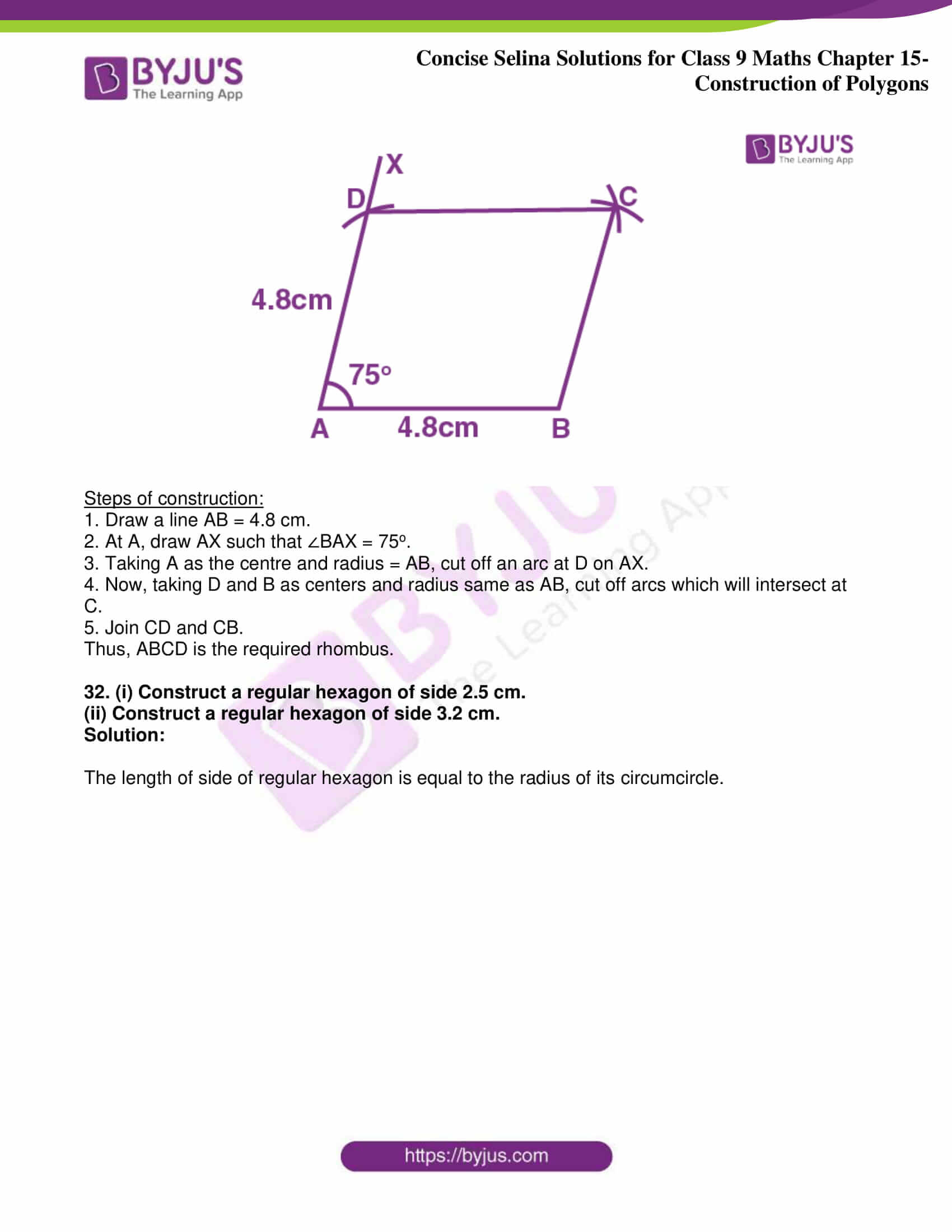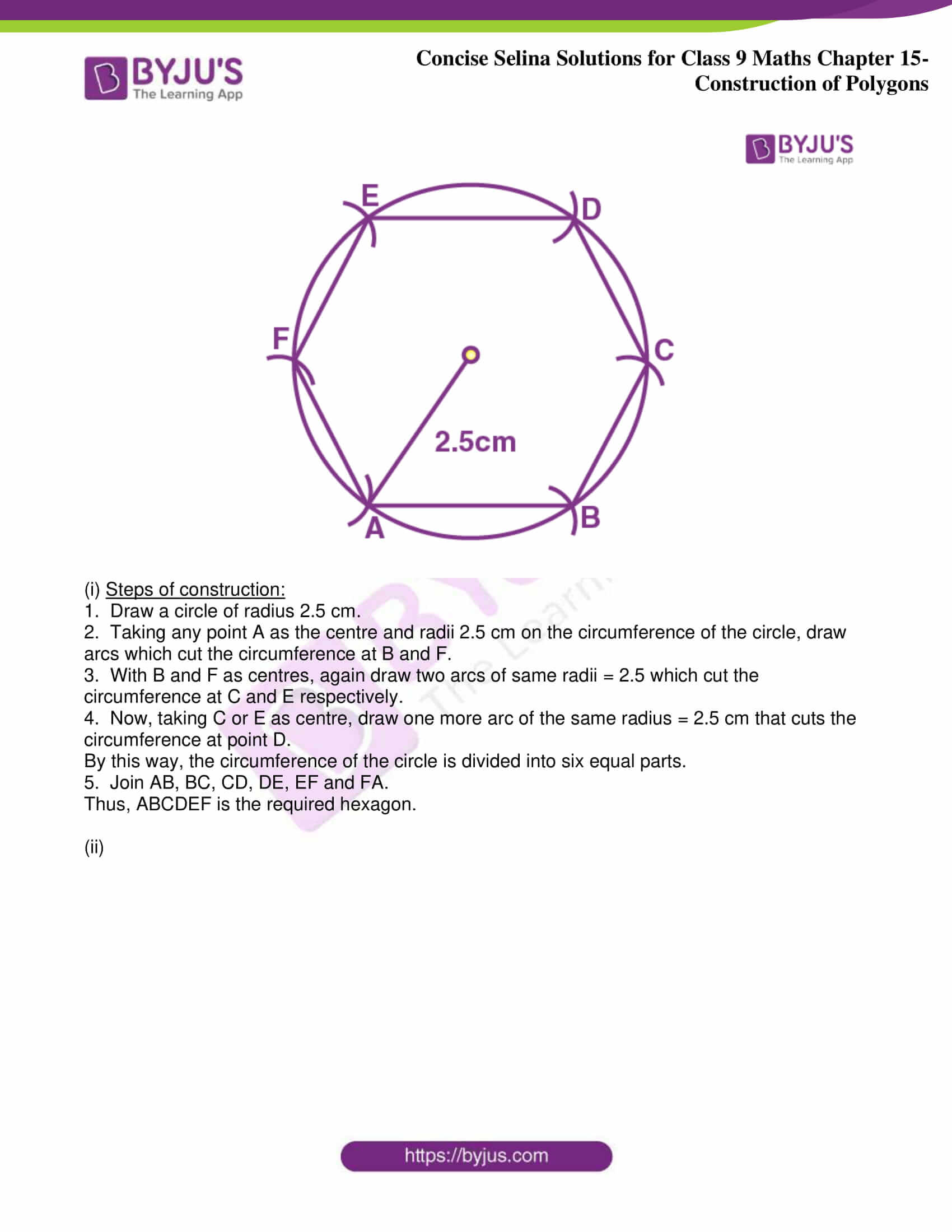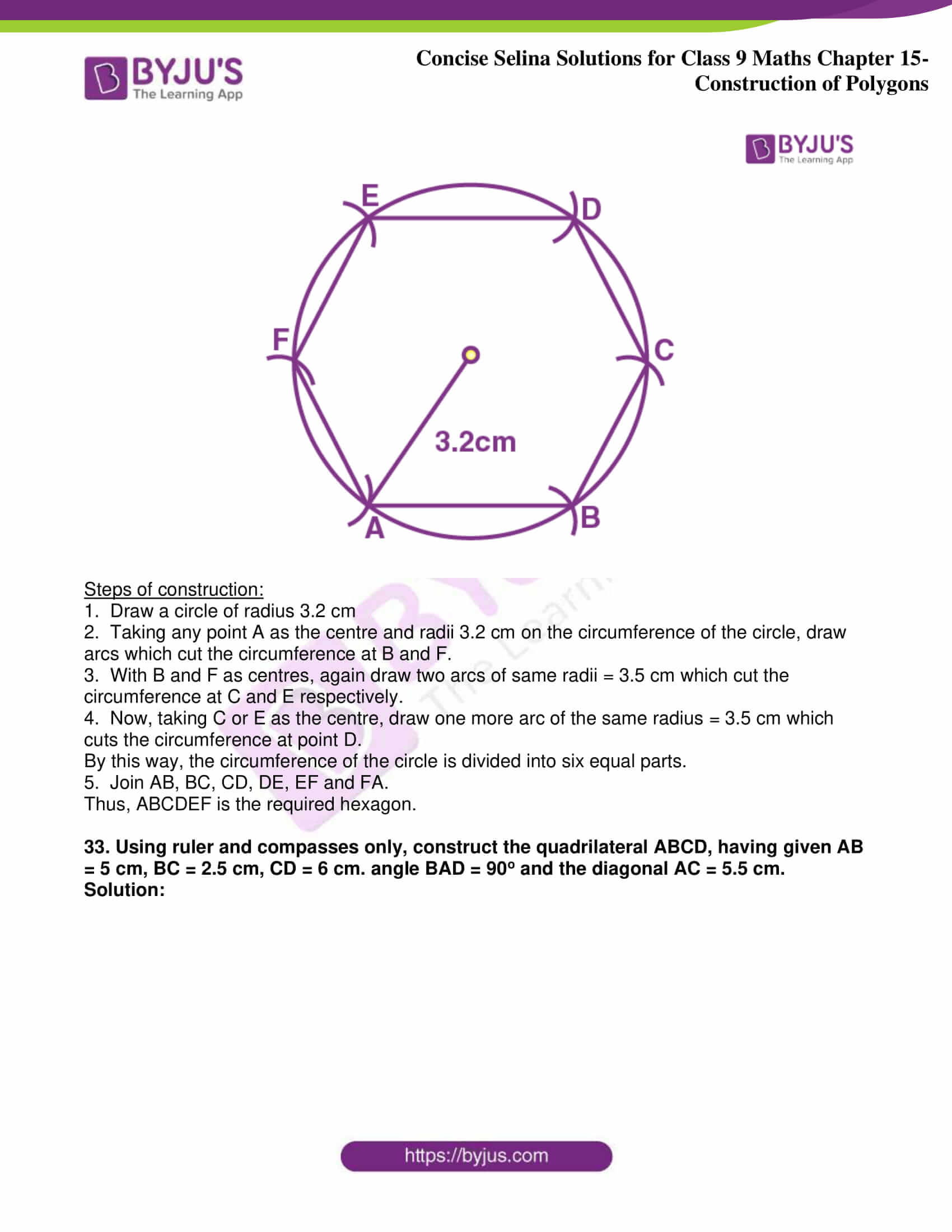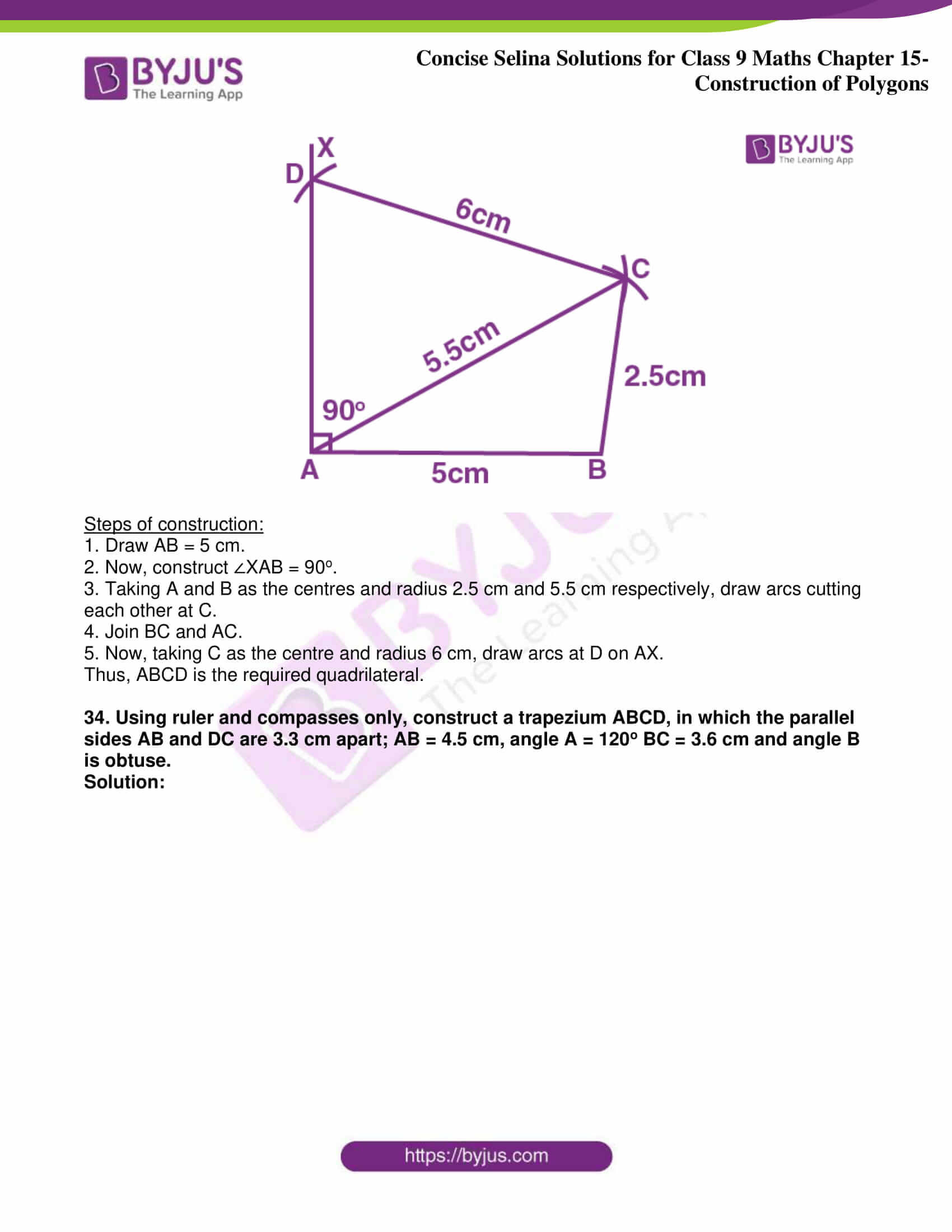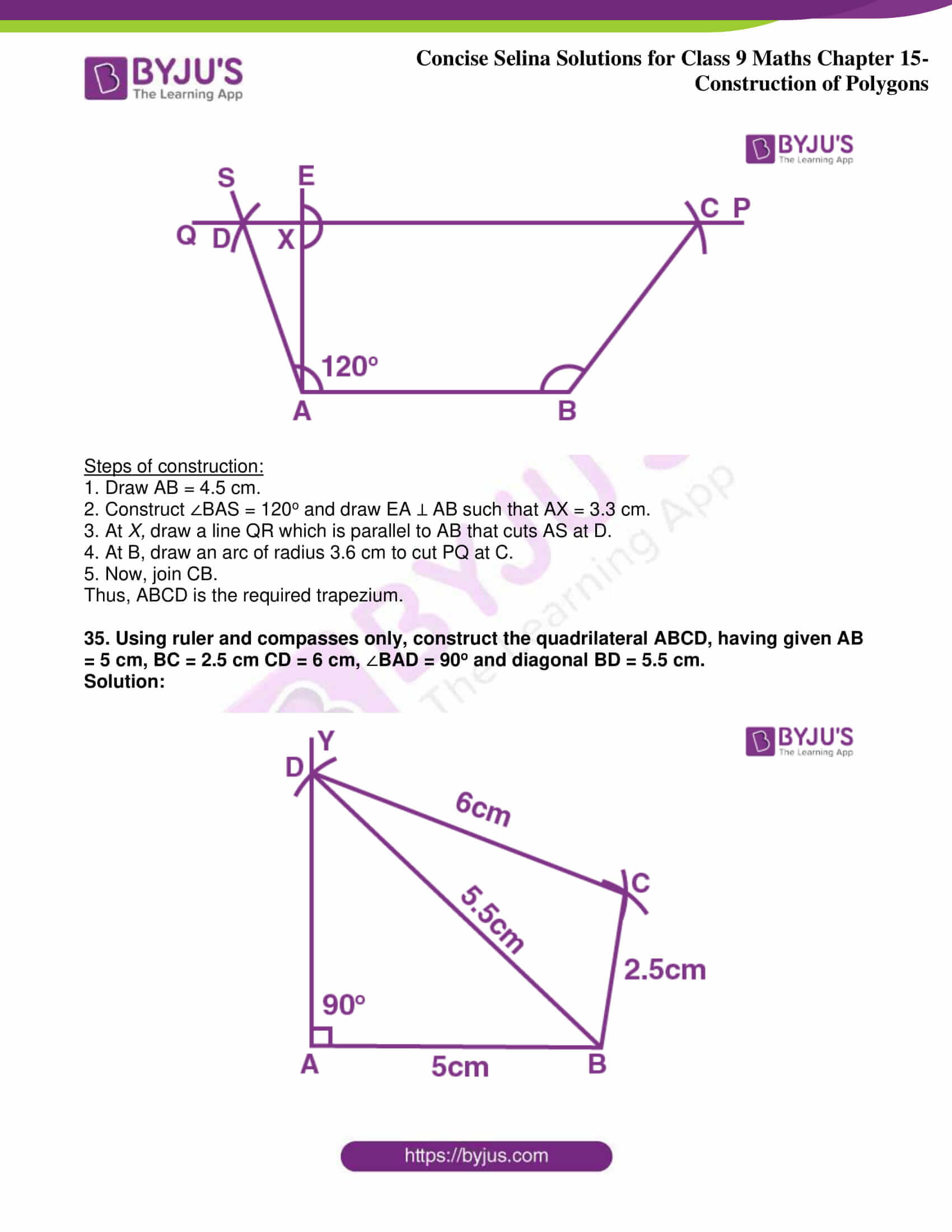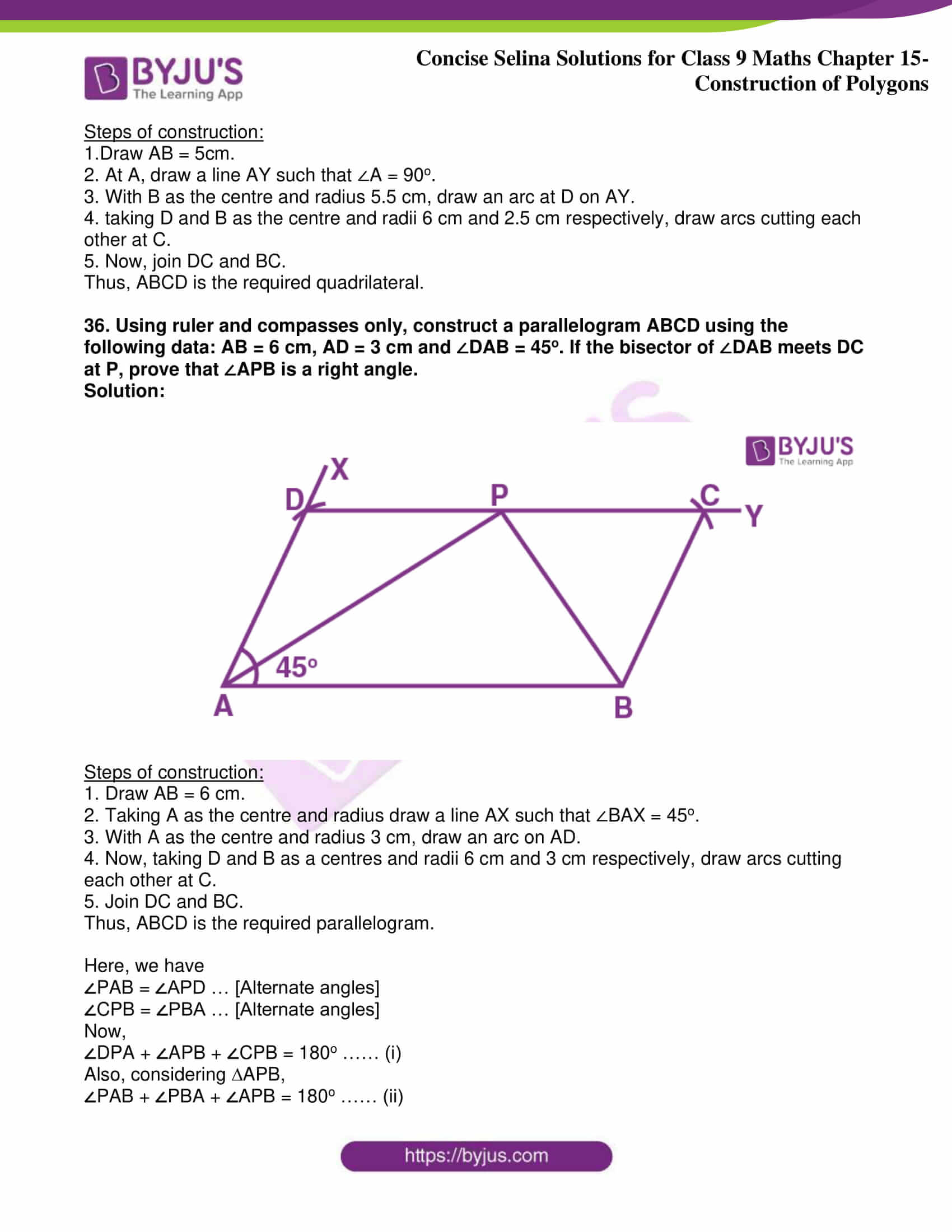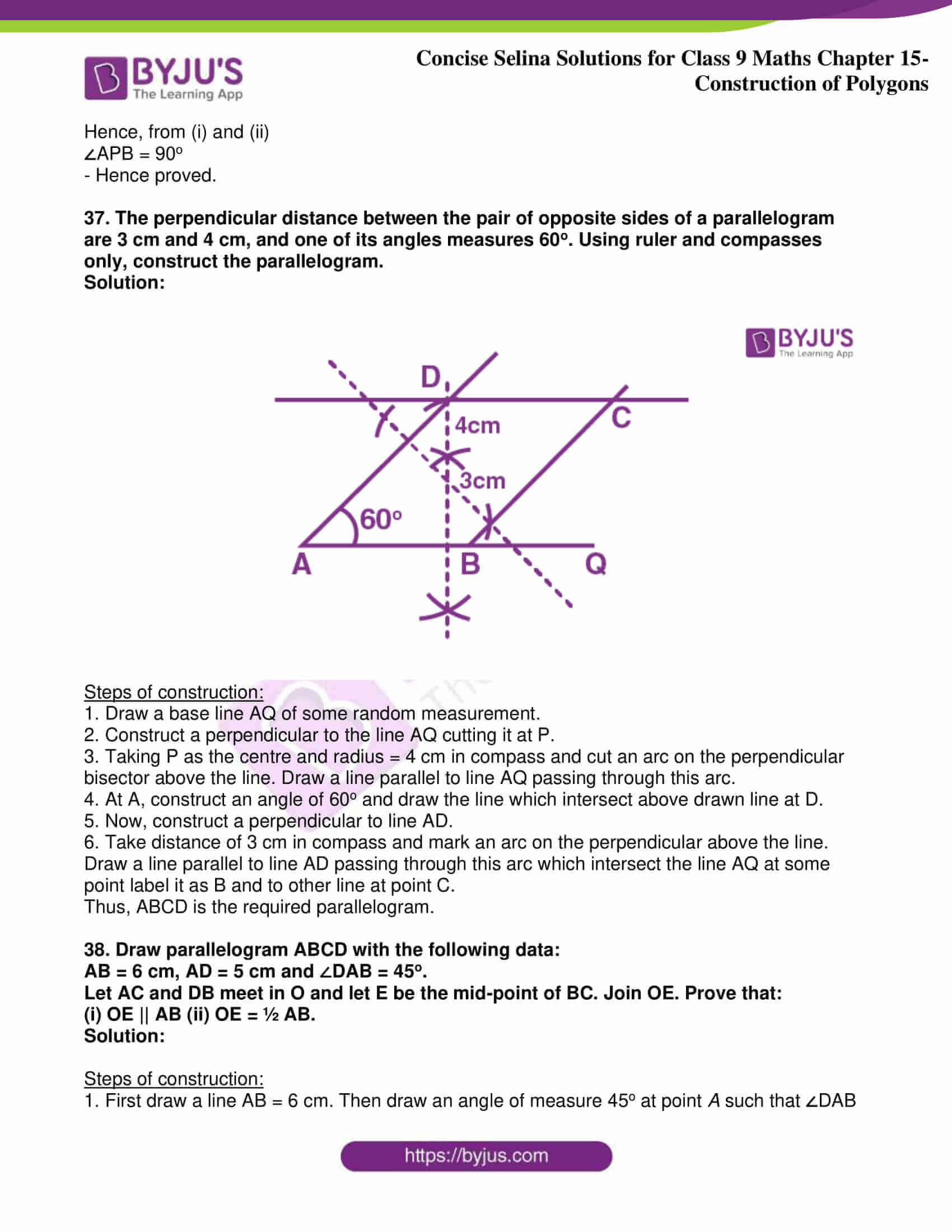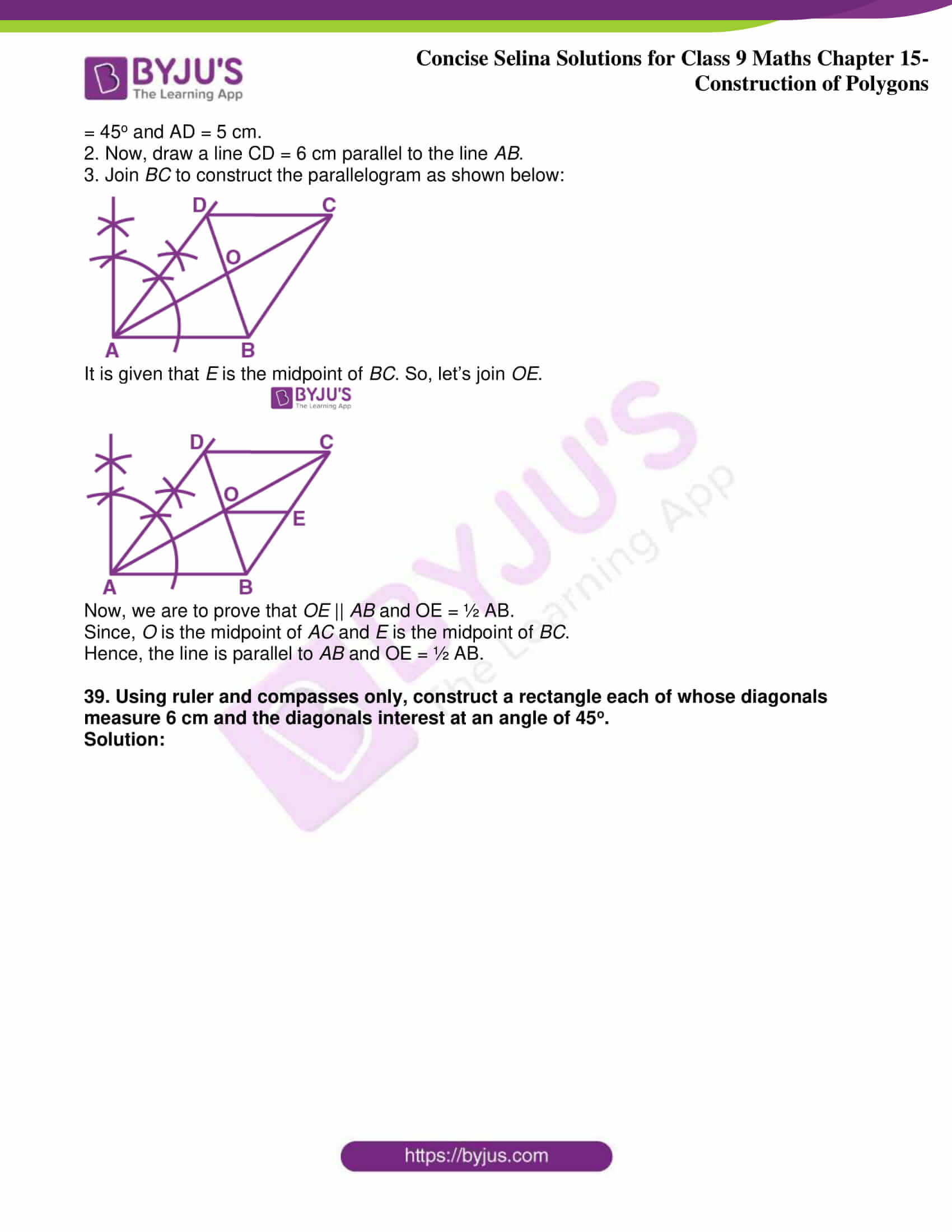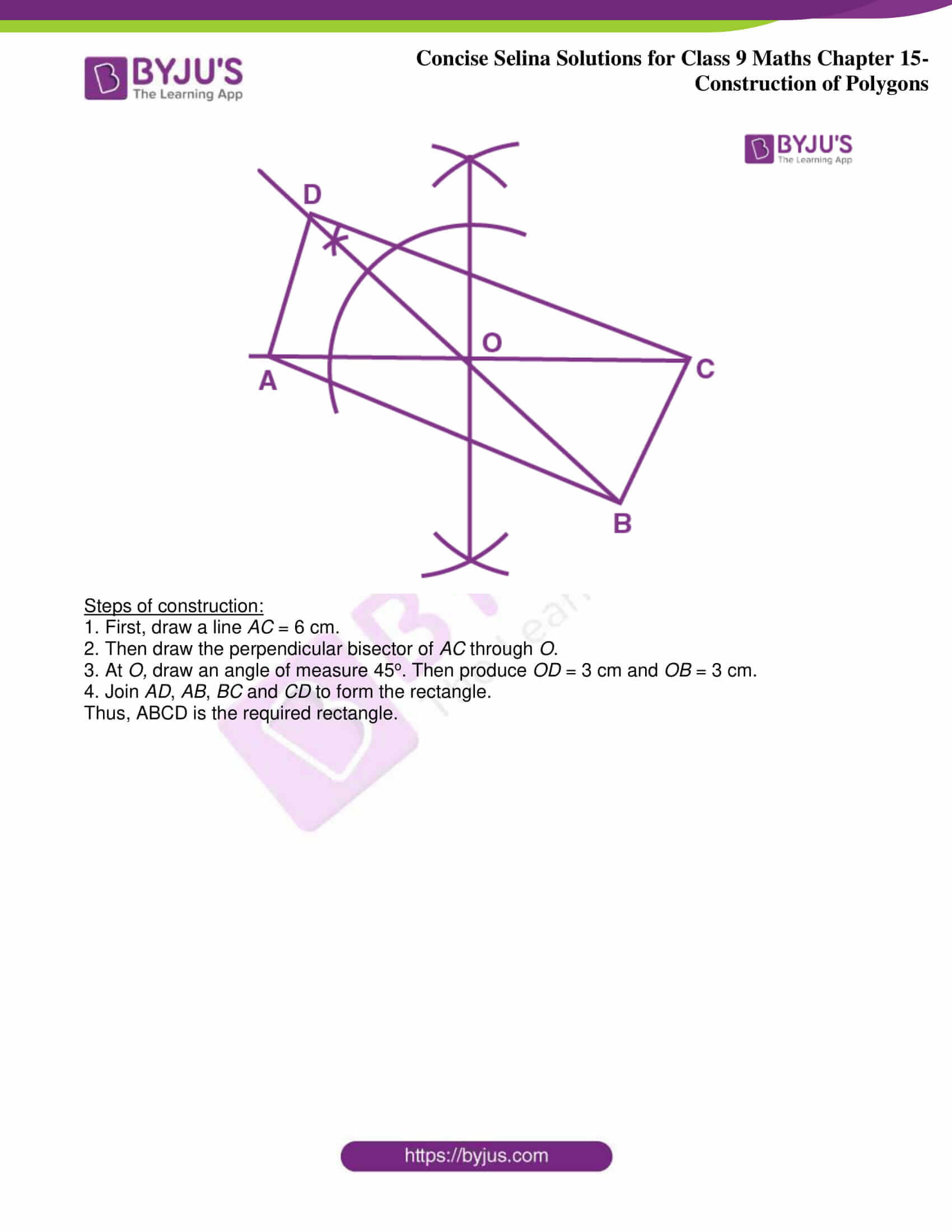Exercise 15

1. AB = 3.2 cm, BC = 5.2 cm, CD = 6.2 cm, DA = 4.2 cm and BD = 5.2 cm.

Solution: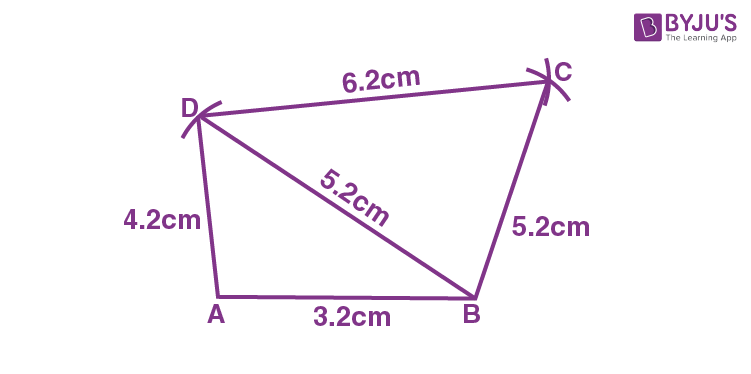Steps of construction:

1. Draw AB = 3.2 cm.

2. With A as the centre and radius 4.2 cm, draw an arc at D and with B as the centre and radius 5.2 cm draw an arc to intersect at D.

3. Now, join AD and DB.

4. With D and B as centres and radii 6.2 cm and 5.2 cm respectively, draw arcs cutting each other at C.

5. Lastly, join BC and DC.

Thus, ABCD is the required quadrilateral.

2. AB = 7.2 cm, BC = 5.8 cm, CD = 6.2 cm, AD = 4.3 cm and angle A = 75o.

Solution: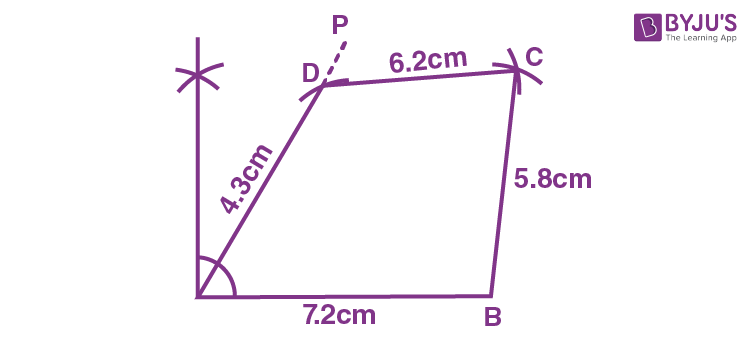Steps of construction:

1. Draw AB = 7.2 cm.

2. At A construct AP such that ∠A = 75o.

3. Cut off AD = 4.3 cm from AP.

4. With D and B as centres and radii 6.2 cm and 5.8 cm respectively, draw arcs cutting each other at C.

5. Now, join DC and BC.

Thus, ABCD is the required quadrilateral.

3. Angle A = 90o, AB = 4.6 cm, BD = 6.4 cm, AC = 6.0 cm and CD = 4.2 cm.

Solution: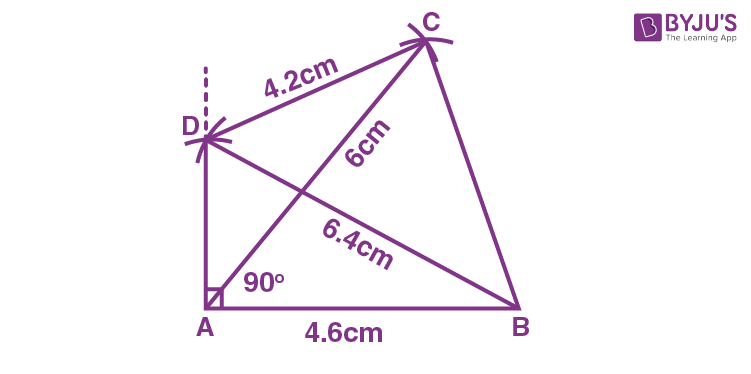Steps of construction:

1. Draw AB = 4.6 cm.

2. At A, construct AP such that ∠A = 90°.

3. With B as the centre and radius 6.4 cm, draw an arc intersecting AP at D.

4. Taking D and A as centres and radii 4.2 cm and 6 cm respectively, draw arcs cutting each other at C.

5. Now, join BD, AC and CB.

Thus, ABCD is the required quadrilateral.

4. AB = 3.8 cm, AC = 4.8 cm, AD = 2.8 cm, angle A = 105o and angle B = 60o.

Solution: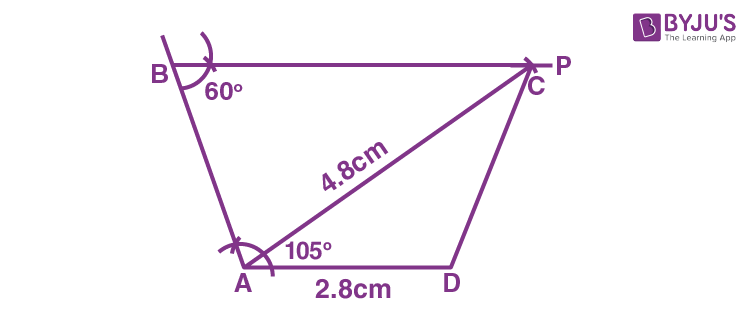Steps of construction:

1. Draw AD = 2.8 cm.

2. Construct AB = 3.8 cm such that ∠A = 105o.

3. Draw BP such that ∠B = 60o.

4. Now, taking A as the centre and radius 4.8 cm, draw an arc cutting BP at C.

Thus, ABCD is the required quadrilateral.

5. BC = 7.5 cm AC = 5.8 cm, AD = 3.6 cm, CD = 4.2 cm and angle A = 120o.

Solution: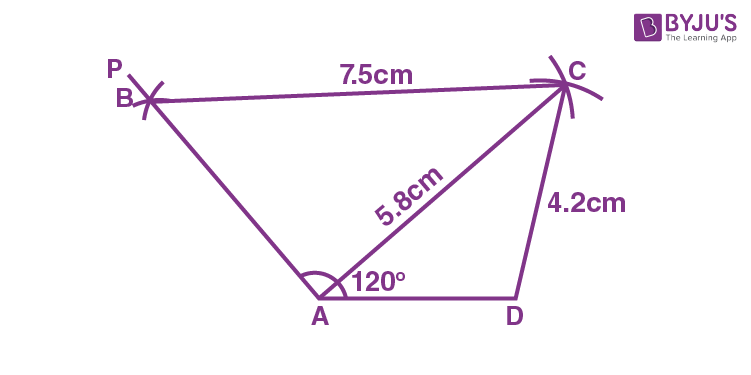Steps of construction:

1. Draw AD = 3.6 cm.

2. Construct AP such that ∠A = 120o.

3. Taking A and D as the centres and radii 5.8 cm and 4.2 cm, draw arcs cutting each other at C.

4. Join AC and CD.

5. Now, taking C as the centre and radius 7.5 cm, draw an arc to intersect AP at B.

6. Join CB.

Thus, ABCD is the required quadrilateral.

6. AD = AB = 4 cm, BC = 2.8 cm, CD = 2.5 cm and angle BAD = 45o.

Solution: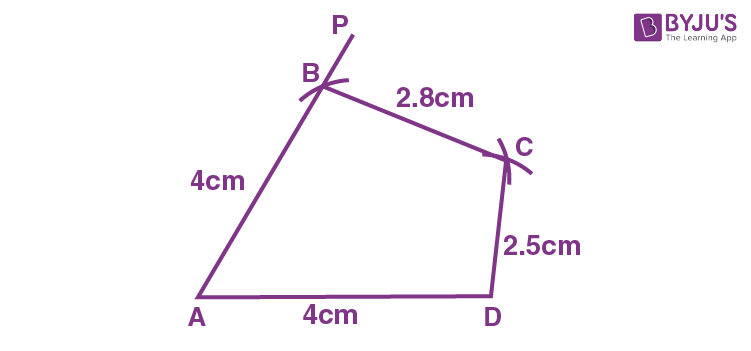Steps of construction:

1. Draw AD = 4 cm.

2. Construct AP such that ∠A = 45o.

3. Taking A as the centre with radius 4 cm, draw an arc cutting AP at B.

4. Now, with B and D as a centres and radii 2.8 cm and 2.8 cm respectively, draw arcs cutting each other at C.

5. Join BC and CD.

Thus, ABCD is the required quadrilateral.

7. AB = 6.3 cm, BC = CD = 4.2 cm and ∠ABC = ∠BCD = 90o.

Solution: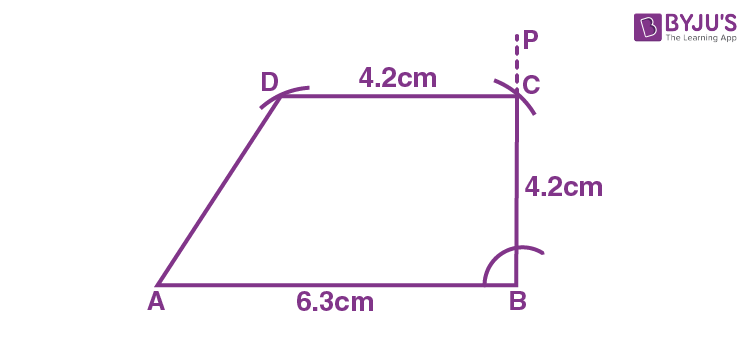Steps of construction:

1. Draw AB = 6.3 cm.

2. Construct BP such that ∠ABP = 90o.

3. Taking B as the centre and radius 4.2 cm, draw an arc to cut AP at C.

4. Now, with C as the centre and radii 4.2 cm, construct CD such that ∠BCD = 90o.

Thus, ABCD is the required quadrilateral.

Construct a parallelogram ABCD, when:

8. AB = 4.4 cm, AD = 6.2 cm and AC = 4.8 cm.

Solution: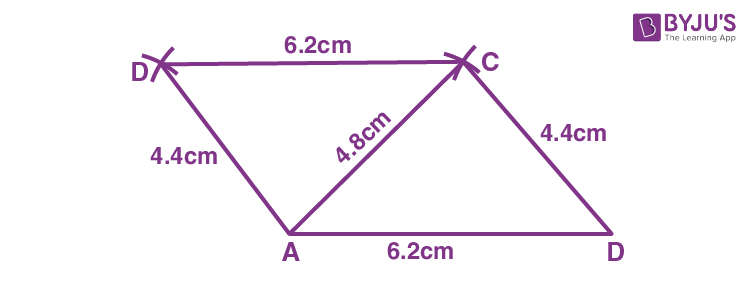Steps of construction:

1. Draw AD = 6.2 cm.

2. Taking A and D as a centres and radii 4.8 cm and 4.4 cm respectively, draw arcs cutting each other at C.

3. Join AC and CD.

4. Now, with A and C as a centres and radii 4.4 cm and 6.2 cm respectively, draw arcs cutting each other at B.

5. Join AB and BC.

Thus, ABCD is the required parallelogram.

9. Diagonal AC = 6.4 cm, diagonals BD = 8.2 cm and angle between the diagonals = 60o.

Solution: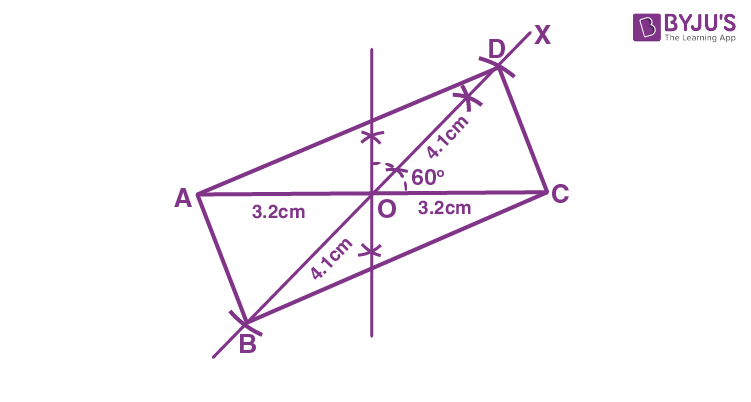Steps of construction:

1. Draw AC = 6.4 cm.

2. Construct BOD such that ∠DOC = 60o and OB = OD = ½ BD = ½ x 8.2 = 4.1 cm.

3. Now, join AB, BC, CD and DA.

Thus, ABCD is the required parallelogram.

10. AB = 5.8 cm, diagonal AC = 8.2 cm and diagonal BD = 6.2 cm.

Solution: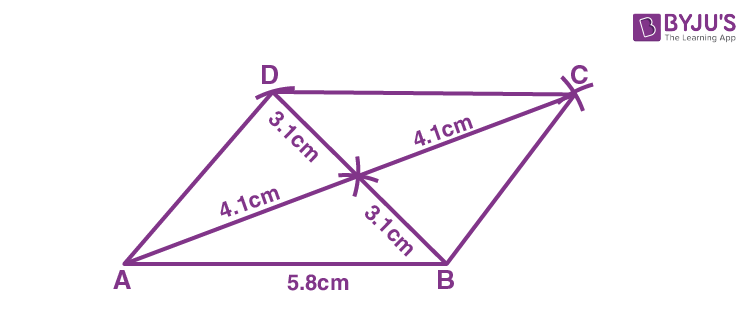Steps of construction:

1. Draw AB = 5.8 cm.

2. As the diagonals of a parallelogram bisect each other.

Construct OAB such that:

OA = ½ AC = ½ x 8.2 cm = 4.1 cm

OB = ½ BD = ½ x 6.2 cm = 3.1 cm

2. Produce AO up to C, such that OC = OA = 4.1 cm and BO up to D, such that DO = OB = 3.1 cm.

3. Now, join AD, DC and CB.

Thus, ABCD is the required parallelogram.

11. AB = 6.0 cm, AD = 5.0 cm and ∠A = 45o.

Solution: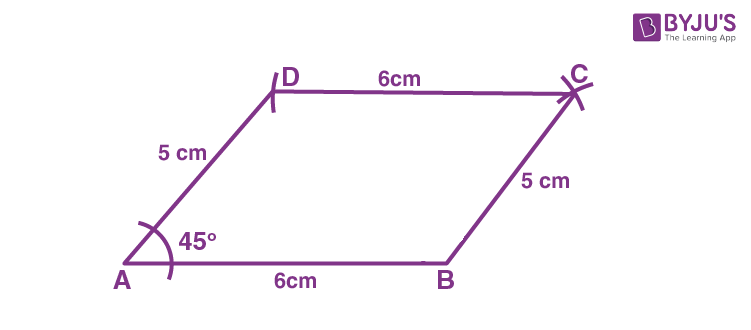Steps of construction:

1. Draw AB = 6 cm.

3. Taking D and B as the centres and radii 6 cm and 5 cm, draw arcs cutting each other at C.

4. Now, join DC and BC.

Thus, ABCD is the required parallelogram.

12. Base AB = 6.5 cm, BC = 4 cm and the altitude corresponding to AB = 3.1 cm.

Solution: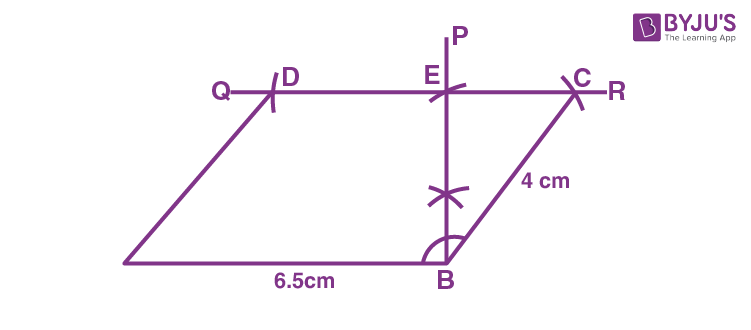Steps of construction:

1. Draw AB = 6.5 cm.

2. At B, construct BP ⊥ AB.

3. Cut off BE = 3.1 cm from BP.

4. At E, construct a perpendicular to BP to obtain QR parallel to AB.

5. Now, taking B as the centre and radius 4 cm, draw an arc which cuts QR at C.

6. With A as a centre and radius 4 cm, draw an arc which cuts QR at D.

Thus, ABCD is the required parallelogram.

13. AB = 4.5 cm, ∠B = 120o and the distance between AB and DC = 3.0 cm.

Solution: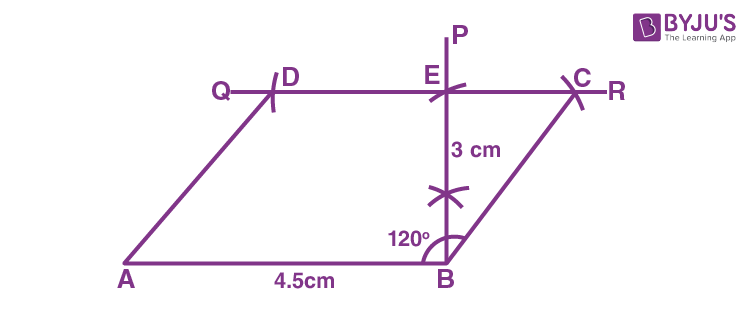Steps of construction:

1. Draw AB = 4.5 cm.

2. At B, construct BP ⊥ AB.

3. Cut off BE = 3 cm, from BP.

4. At E, draw a perpendicular to BP to obtain QR parallel to AB.

5. Now, taking B as the centre draw an arc which cuts QR at C such that ∠B = 120o.

6. With A as a centre and radius BC, draw an arc which cuts QR at D.

Thus, ABCD is the required parallelogram.

14. Base BC = 5.6 cm, diagonal BD = 6.5 cm and altitude = 3.2 cm.

Solution: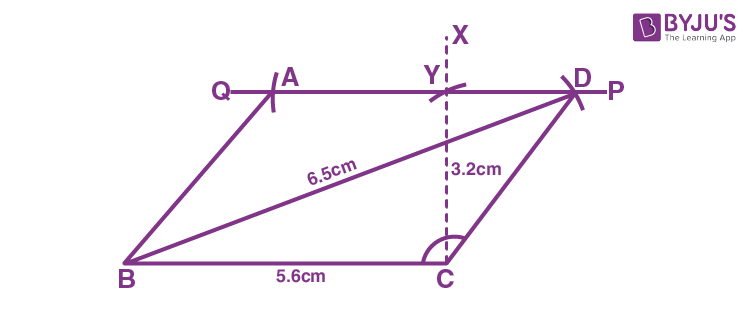Steps of construction:

1. Draw BC = 5.6 cm.

2. At C, construct CX ⊥ BC.

3. Taking C as the centre and radius 3.2 cm draw an arc to cut CX at Y.

4. At Y, draw a straight-line PQ parallel to BC.

5. Now, taking B as the centre and radius 6.5 cm, draw an arc to meet PQ at D.

6. With D as a centre and radius to 5.6 cm, draw an arc to meet PQ at A.

7. Join BA, BD and CD.

Thus, ABCD is the required parallelogram.

Construct a rectangle ABCD, when

15. Its sides are 6.0 cm and 7.2 cm.

Solution:

We know that, each angle of a rectangle is 90o and opposite sides are equal.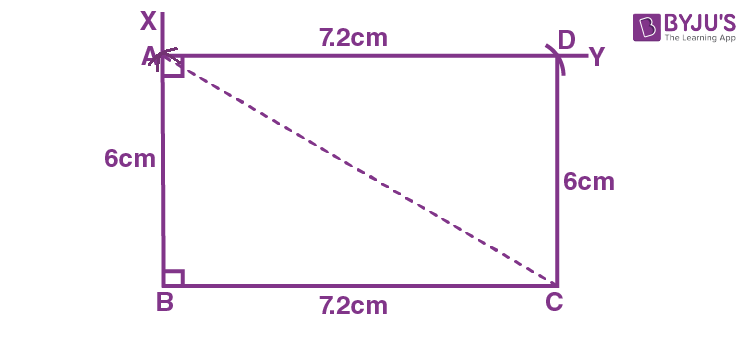Steps of construction:

1. Draw BC = 7.2 cm.

2. Taking B as the centre, draw a line BX such that ∠B = 90o.

3. Now, with B as the centre and radius 6 cm draw an arc to cut BX at A.

4. At A, construct a line AY parallel to BC.

5. Taking A as the centre and radius 7.2 cm draw an arc to cut AY at D.

6. Join CD.

Thus, ABCD is the required rectangle.

16. One side = 4 cm and one diagonal is 5 cm. Measure the length of other side.

Solution: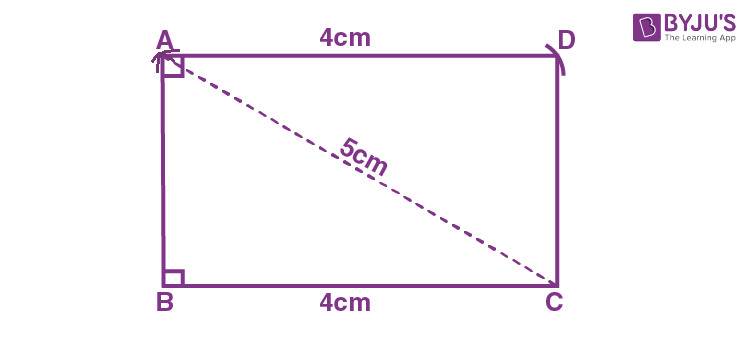Steps of construction:

1. Draw BC = 4 cm.

2. Taking C as the centre and radius 5 cm, draw an arc at A such that ∠ABC = 90o.

3. Join AB and AC.

4. Now, with A as a centre and radius 4 cm, draw an arc at D such that ∠ADC = 90o.

Thus, ABCD is the required rectangle.

17. One diagonal = 6.0 cm and the acute angle between the diagonals = 45o.

Solution: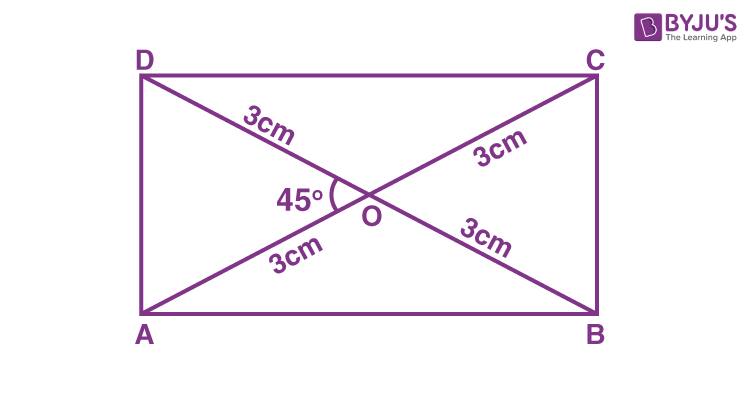Steps of construction:

1. Draw AC = 6 cm.

2. Now, bisect AC at O.

3. At O, construct ∠XOC = 45° and produce XO to Y.

4. Cut OB = OD = 3 cm (i.e., half the diagonal 6 cm)

5. Join AB, CB, AD and CD.

Thus, ABCD is the required rectangle

18. Area = 24 cm2 and base = 4.8 cm.

Solution:

Given,

Base = 4.8 cm and area = 24 cm2

We know that, area of rectangle = base x height

So,

24 = 4.8 x height

Height = 24/4.8 = 5 cm

The rectangle with base = 4.8 cm and height = 5 cm is constructed as below.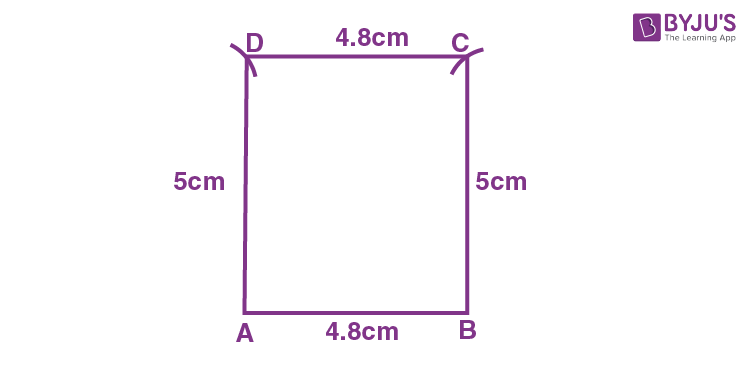Steps of construction:

1. Draw base, AB = 4.8 cm.

2. Taking A and B as a centres and radii 5 cm each, draw arcs at D and C.

3. Now, join AD, BC and DC.

Thus, ABCD is the required rectangle.

19. Area = 36 cm2 and height = 4.5 cm.

Solution:

Given,

Height = 4.5 cm and area = 36 cm2

We know that, area of rectangle = base x height

So,

36 = base x 4.5

Base = 36/4.5 = 8 cm

The rectangle with base = 8 cm and height = 4.5 cm is constructed as below.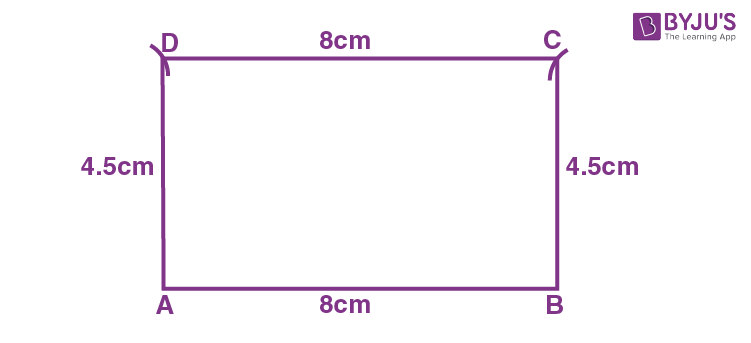Steps of construction:

1. Draw base, AB = 8 cm.

2. Taking A and B as a centres and radii 4.5 cm each, draw arcs at D and C.

3. Join AD, BC and DC.

Thus, ABCD is the required rectangle.

Construct a trapezium ABCD, when:

20. AB = 4.8 cm, BC = 6.8 cm, CD = 5.4 cm, angle B = 60o and AD || BC.

Solution: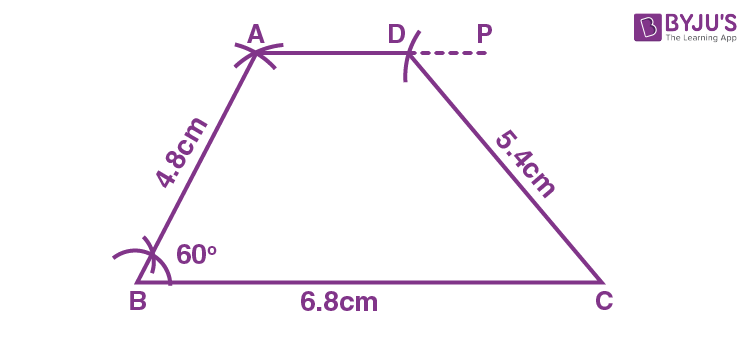Steps of construction:

1. Draw BC = 6.8 cm.

2. Taking B as the centre and radius 4.8 cm, draw an arc at A such that ∠B = 60o.

3. At A, draw a line AP such that AP || BC.

4. With C as the centre and radius 5.4 cm, draw an arc AP to cut at D.

5. Now, join AB and CD.

Thus, ABCD is the required trapezium.

21. AB = CD = 3.2 cm, BC = 6.0 cm, AD = 4.1 cm and AD // BC.

Solution: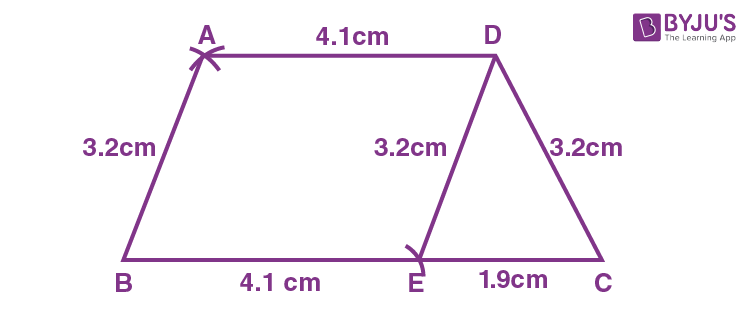Steps of construction:

1. Draw BC = 6 cm.

2. Cut off BE = AD = 4.1 cm from BC.

3. Construct triangle DEC such that DE = AB = 3.2 cm and CD = 3.2 cm.

4. Taking B and D as the centres and radii 3.2 cm and 4.1 cm respectively, draw arcs cutting each other at A.

Thus, ABCD is the required trapezium.

Construct a rhombus ABCD, when:

22. It’s one side = 6 cm and A = 60o.

Solution: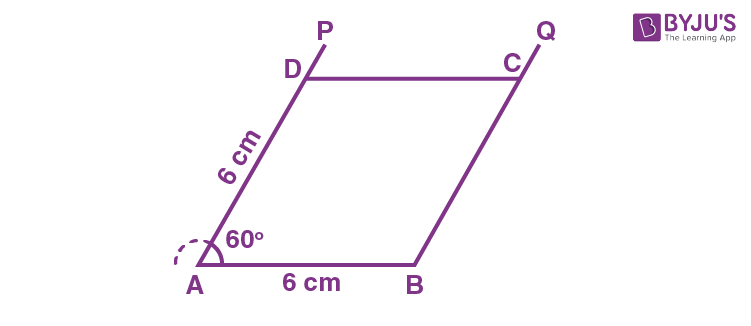Steps of construction:

1. Draw AB = 6 cm.

2. At A, construct ∠BAP = 60o.

3. From AP, cut off D such that AD = 6 cm.

4. At B, construct BQ || AD.

5. At D, construct DC || AB to cut BQ at C.

Thus, ABCD is the required rhombus.

23. One side = 5.4 cm and one diagonals is 7.0 cm.

Solution: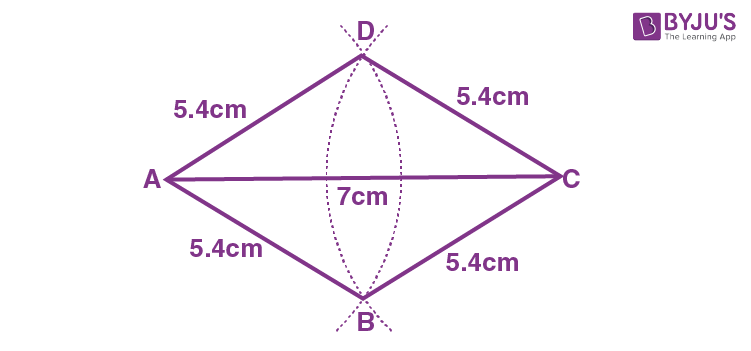Steps of construction:

1. Draw AC = 7 cm.

2. Taking A as the centre and radius 5.4 cm, draw an arc extending on both sides of AC.

3. Taking C as the centre and radius 5.4 cm, draw an arc extending on both sides of AC to cut the first arc at B and D.

4. Now, join AB, BC, CD and DA.

Thus, ABCD is the required rhombus.

24. Diagonal AC = 6.3 cm and diagonal BD = 5.8 cm.

Solution: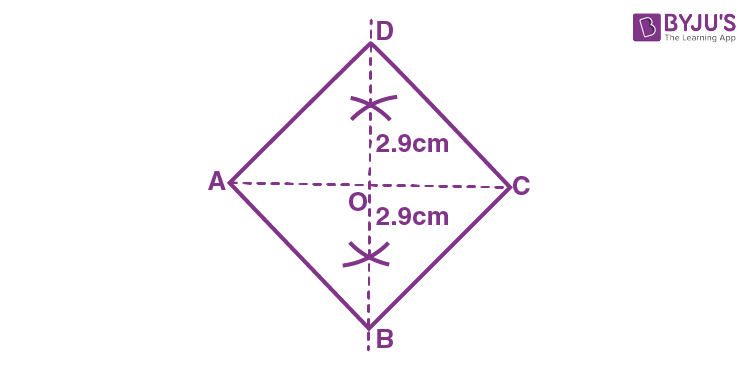Steps of construction:

1. Draw AC = 6.3 cm.

2. Construct the perpendicular bisector of AC which cuts AC at O.

3. Cut off OD and OB on the perpendicular bisector such that,

OD = OB = ½ BD = ½ x 5.8 = 2.9 cm

4. Join AB, BC, CD and DA.

Thus, ABCD is the required rhombus.

25. One side = 5.0 cm and height = 2.6 cm.

Solution: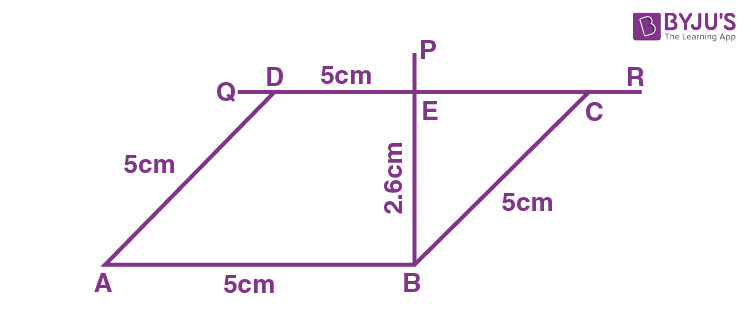Steps of construction:

1. Draw AB = 5 cm.

2. At B, construct BP ⊥ AB.

3. Cut off BE = 2.6 cm from BP.

4. At E, draw perpendicular to CP so that QR || AB.

5. Taking A and B as the centres and radii 5 cm each, draw arcs cutting QR at D and C.

Thus, ABCD is the required rhombus.

26. A = 60o and height = 3.0 cm.

Solution: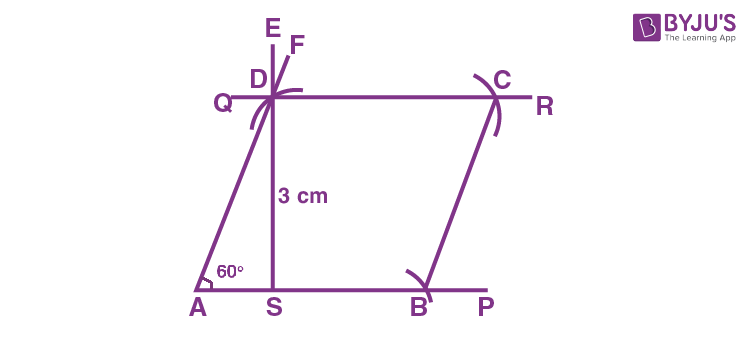Steps of construction:

1. Draw a line AP.

2. Now, draw AF such that ∠A = 60o.

3. At S, construct a perpendicular SE = 3 cm such that it cuts AF at D.

4. Through D draw a line QR parallel to AP.

5. Taking the radius same as AD, draw an arc at B on AP.

6. Now, at B taking radius same as AD and AB, draw arcs cutting each other at C.

7. Join BC.

Thus, ABCD is the required rhombus.

27. Diagonal AC = 6.0 cm and height = 3.5 cm.

Solution: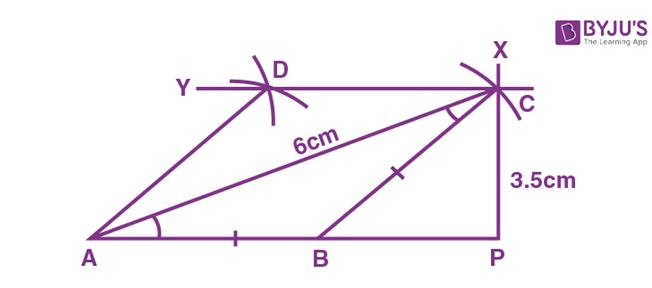Steps of construction:

1. Draw a line AP.

2. Taking A and P as the centres and radii 6 cm and 3.5 cm respectively, draw arcs to intersect each other at C.

3. Now, draw BC such that AB = BC.

4. At C, draw a line CY parallel to AP.

5. At points C and A, taking radius same as AB, draw arcs cutting each other at D.

Thus, ABCD is the required rhombus.

Construct a square ABCD, when:

28. One side = 4.5 cm.

Solution: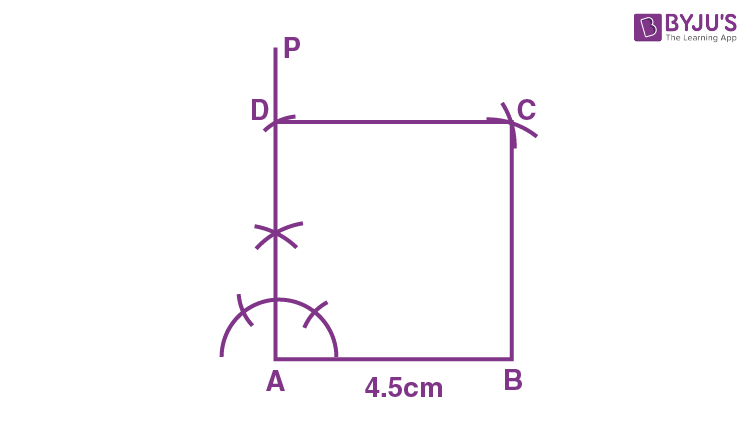Steps of construction:

1. Draw AB = 4.5 cm.

2. Construct AP ⊥ AB.

3. Cut off AD = 4.5 cm, from AP.

4. Taking B as the centre and radius 4.5 cm, draw an arc.

5. Now, with D as the centre and radius 4.5 cm, draw another arc cutting the previous arc at C.

6. Join BC and CD.

Thus, ABCD is the required square.

29. One diagonal = 5.4 cm.

Solution:

We know that, the diagonals of a square are equal and bisect each other at right angles.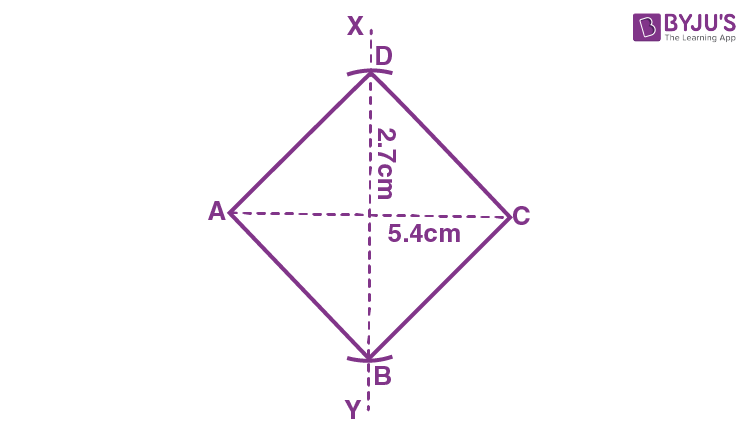Steps of construction:

1. Draw AC = 5.4 cm.

2. Construct the right bisector XY of AC, meeting AC at O.

3. Now, from O, set off OB = ½ (5.4) = 2.7 cm along OY and OD = 2.7 cm along OX.

4. Join AB, BC, CD and DA.

Thus, ABCD is the required square.

30. Construct a square ABCD, when perimeter = 24 cm.

Solution:

Given, perimeter of the square = 24 cm

We know that,

Perimeter of square, P = 4a

Where, a is the length of each side.

So,

24 = 4a

a = 24/4 = 6 cm

Therefore, the sides of the squares are of length 6 cm.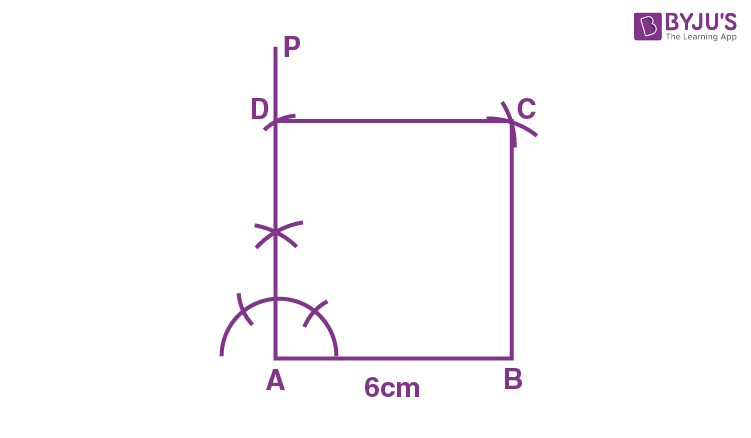Steps of construction:

1. Draw AB = 6cm.

2. Construct AP ⊥ AB.

3. Cut off AD = 6 cm from AP.

4. Taking B as the centre and radius 6 cm, draw an arc.

5. Now, with D as the centre and radius 6 cm, draw another arc cutting the previous arc at C.

6. Join BC and CD.

Thus, ABCD is the required square.

31. Construct a rhombus, having given one side = 4.8 cm and one angle = 75o.

Solution: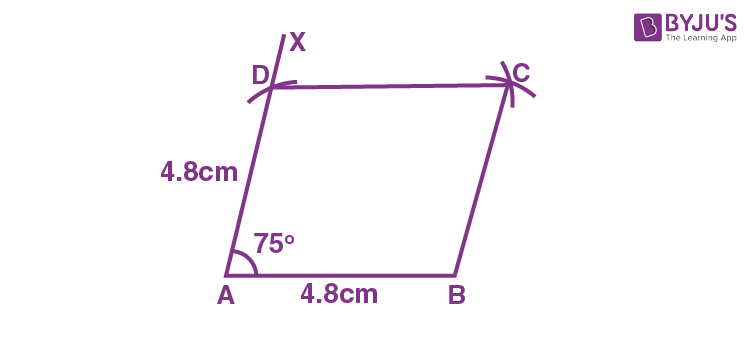Steps of construction:

1. Draw a line AB = 4.8 cm.

2. At A, draw AX such that ∠BAX = 75o.

3. Taking A as the centre and radius = AB, cut off an arc at D on AX.

4. Now, taking D and B as centers and radius same as AB, cut off arcs which will intersect at C.

5. Join CD and CB.

Thus, ABCD is the required rhombus.

32. (i) Construct a regular hexagon of side 2.5 cm.

(ii) Construct a regular hexagon of side 3.2 cm.

Solution:

The length of side of regular hexagon is equal to the radius of its circumcircle.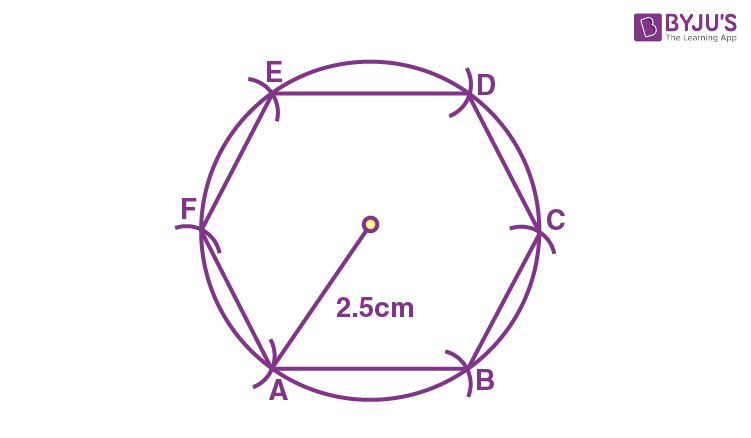(i) Steps of construction:

1.  Draw a circle of radius 2.5 cm.

2.  Taking any point A as the centre and radii 2.5 cm on the circumference of the circle, draw arcs which cut the circumference at B and F.

3.  With B and F as centres, again draw two arcs of same radii = 2.5 which cut the circumference at C and E respectively.

4.  Now, taking C or E as centre, draw one more arc of the same radius = 2.5 cm that cuts the circumference at point D.

By this way, the circumference of the circle is divided into six equal parts.

5.  Join AB, BC, CD, DE, EF and FA.

Thus, ABCDEF is the required hexagon.

(ii)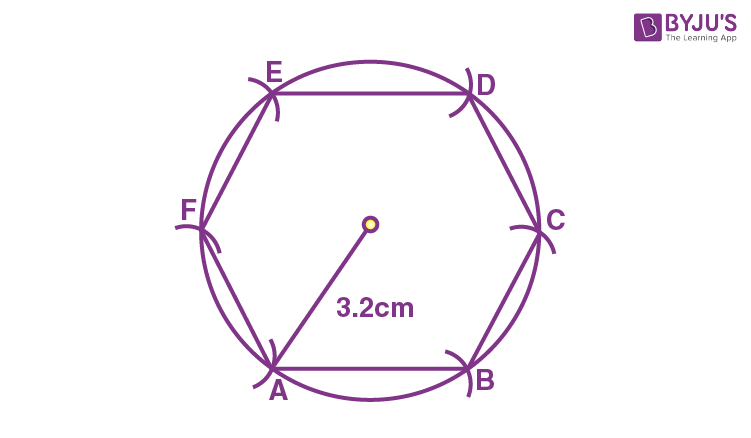Steps of construction:

1.  Draw a circle of radius 3.2 cm

2.  Taking any point A as the centre and radii 3.2 cm on the circumference of the circle, draw arcs which cut the circumference at B and F.

3.  With B and F as centres, again draw two arcs of same radii = 3.5 cm which cut the circumference at C and E respectively.

4.  Now, taking C or E as the centre, draw one more arc of the same radius = 3.5 cm which cuts the circumference at point D.

By this way, the circumference of the circle is divided into six equal parts.

5.  Join AB, BC, CD, DE, EF and FA.

Thus, ABCDEF is the required hexagon.

33. Using ruler and compasses only, construct the quadrilateral ABCD, having given AB = 5 cm, BC = 2.5 cm, CD = 6 cm. angle BAD = 90o and the diagonal AC = 5.5 cm.

Solution: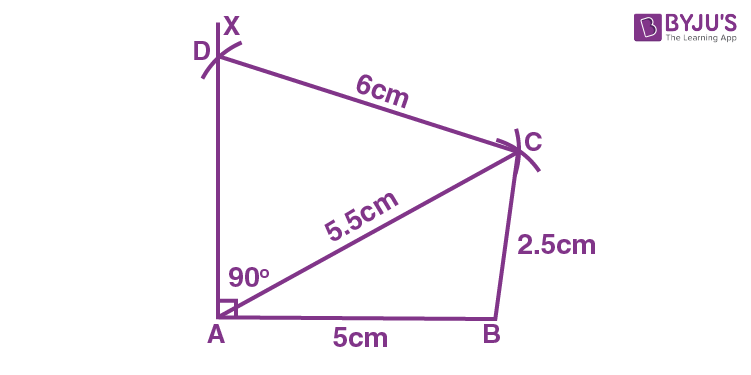Steps of construction:

1. Draw AB = 5 cm.

2. Now, construct ∠XAB = 90o.

3. Taking A and B as the centres and radius 2.5 cm and 5.5 cm respectively, draw arcs cutting each other at C.

4. Join BC and AC.

5. Now, taking C as the centre and radius 6 cm, draw arcs at D on AX.

Thus, ABCD is the required quadrilateral.

34. Using ruler and compasses only, construct a trapezium ABCD, in which the parallel sides AB and DC are 3.3 cm apart; AB = 4.5 cm, angle A = 120o BC = 3.6 cm and angle B is obtuse.

Solution: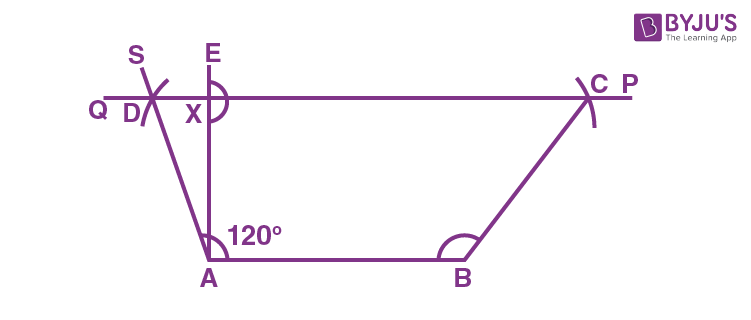Steps of construction:

1. Draw AB = 4.5 cm.

2. Construct ∠BAS = 120o and draw EA ⊥ AB such that AX = 3.3 cm.

3. At X, draw a line QR which is parallel to AB that cuts AS at D.

4. At B, draw an arc of radius 3.6 cm to cut PQ at C.

5. Now, join CB.

Thus, ABCD is the required trapezium.

35. Using ruler and compasses only, construct the quadrilateral ABCD, having given AB = 5 cm, BC = 2.5 cm CD = 6 cm, BAD = 90o and diagonal BD = 5.5 cm.

Solution: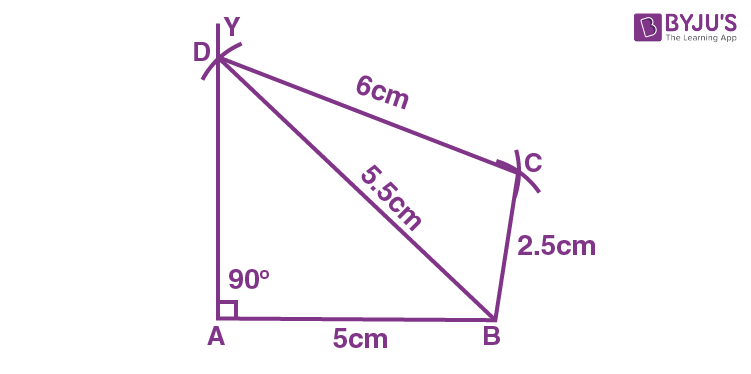Steps of construction:

1.Draw AB = 5cm.

2. At A, draw a line AY such that ∠A = 90o.

3. With B as the centre and radius 5.5 cm, draw an arc at D on AY.

4. taking D and B as the centre and radii 6 cm and 2.5 cm respectively, draw arcs cutting each other at C.

5. Now, join DC and BC.

Thus, ABCD is the required quadrilateral.

36. Using ruler and compasses only, construct a parallelogram ABCD using the following data: AB = 6 cm, AD = 3 cm and ∠DAB = 45o. If the bisector of ∠DAB meets DC at P, prove that ∠APB is a right angle.

Solution: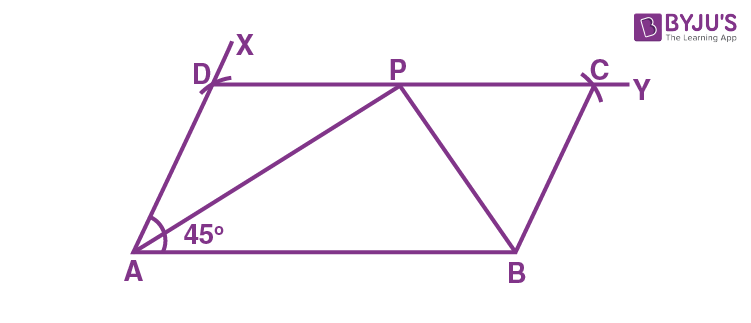Steps of construction:

1. Draw AB = 6 cm.

2. Taking A as the centre and radius draw a line AX such that ∠BAX = 45o.

3. With A as the centre and radius 3 cm, draw an arc on AD.

4. Now, taking D and B as a centres and radii 6 cm and 3 cm respectively, draw arcs cutting each other at C.

5. Join DC and BC.

Thus, ABCD is the required parallelogram.

Here, we have

PAB = APD … [Alternate angles]

CPB = PBA … [Alternate angles]

Now,

DPA + APB + CPB = 180o …… (i)

Also, considering ∆APB,

PAB + PBA + APB = 180o …… (ii)

Hence, from (i) and (ii)

APB = 90o

– Hence proved.

37. The perpendicular distance between the pair of opposite sides of a parallelogram are 3 cm and 4 cm, and one of its angles measures 60o. Using ruler and compasses only, construct the parallelogram.

Solution: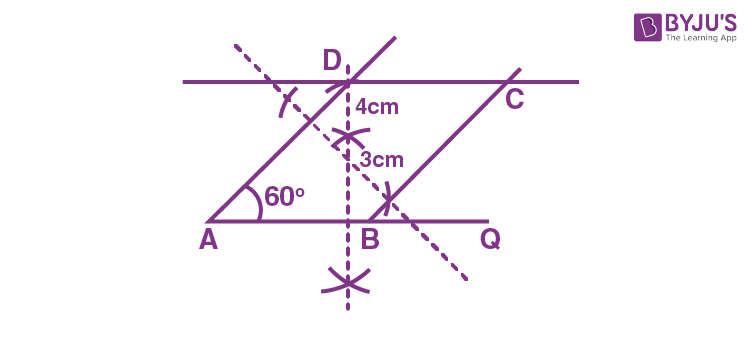Steps of construction:

1. Draw a base line AQ of some random measurement.

2. Construct a perpendicular to the line AQ cutting it at P.

3. Taking P as the centre and radius = 4 cm in compass and cut an arc on the perpendicular bisector above the line. Draw a line parallel to line AQ passing through this arc.

4. At A, construct an angle of 60o and draw the line which intersect above drawn line at D.

5. Now, construct a perpendicular to line AD.

6. Take distance of 3 cm in compass and mark an arc on the perpendicular above the line. Draw a line parallel to line AD passing through this arc which intersect the line AQ at some point label it as B and to other line at point C.

Thus, ABCD is the required parallelogram.

38. Draw parallelogram ABCD with the following data:

AB = 6 cm, AD = 5 cm and ∠DAB = 45o.

Let AC and DB meet in O and let E be the mid-point of BC. Join OE. Prove that:

(i) OE || AB (ii) OE = ½ AB.

Solution:

Steps of construction:

1. First draw a line AB = 6 cm. Then draw an angle of measure 45o at point A such that DAB = 45o and AD = 5 cm.

2. Now, draw a line CD = 6 cm parallel to the line AB.

3. Join BC to construct the parallelogram as shown below: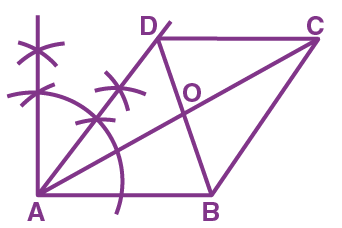It is given that E is the midpoint of BC. So, let’s join OE.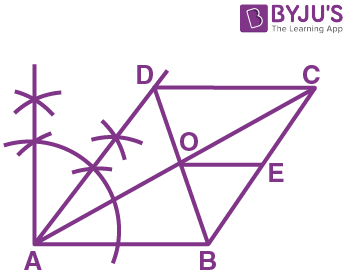Now, we are to prove that OE || AB and OE = ½ AB.

Since, O is the midpoint of AC and E is the midpoint of BC.

Hence, the line is parallel to AB and OE = ½ AB.

39. Using ruler and compasses only, construct a rectangle each of whose diagonals measure 6 cm and the diagonals interest at an angle of 45o.

Solution: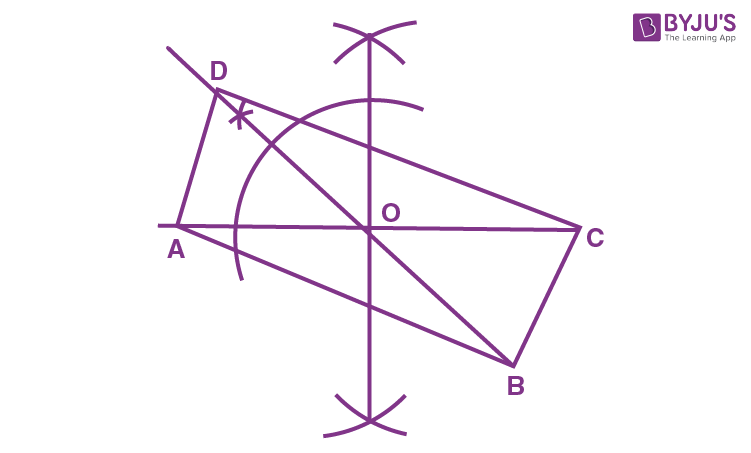Steps of construction:

1. First, draw a line AC = 6 cm.

2. Then draw the perpendicular bisector of AC through O.

3. At O, draw an angle of measure 45o. Then produce OD = 3 cm and OB = 3 cm.

4. Join ADABBC and CD to form the rectangle.

Thus, ABCD is the required rectangle.

## Selina Solutions for Class 9 Maths Chapter 15- Construction of Polygons

The Chapter 15, Construction of Polygons, contains 1 exercise and the Selina Solutions given here provides the answers for all the questions present in this exercise. Let us have a look at some of the topics that are being discussed in this chapter.

15.2 Construction of Parallelograms

15.3 Construction of Trapezium

15.4 Construction of Rectangles

15.5 Construction of Rhombus

15.6 Construction of Square

15.7 Construction of Regular Hexagon

## Selina Solutions for Class 9 Maths Chapter 15- Construction of Polygons

The Chapter 15 of Class 9 Maths Concise Selina textbook helps students in learning the construction of different polygons. From quadrilaterals to parallelograms, rectangles, squares, trapezium etc. Read and learn the Chapter 15 of Selina textbook to learn more about Construction of Polygons along with the concepts covered in it. Solve the Selina Solutions for Class 9 effectively to score high in the examination.